### PyCharm: PyCharm 2019.1.1

PyCharm is the first JetBrains IDE to ship with the new JDK 11. This brings us improved performance and better rendering for our Jupyter Notebooks. Unfortunately, it also means that we ran into a couple of teething issues with the new JDK.

# New in this Version

## AltGr works correctly

As those of you who are using keymaps that require the AltGr key to type various characters have kindly let us know, this ability was broken in PyCharm 2019.1. We published a workaround as soon as we could, and have been making progress to resolving the issue. We heard that the fix we released with the release candidate of this version didn’t resolve the issue for all keyboard layouts, so we’ve revised the fix and verified it to work with all keyboard layouts mentioned in the issues on YouTrack.

## Further Improvements

• Previously, Python 3.8 pre-release interpreters were detected as Python 3.7, they’re now correctly identified as Python 3.8
• Various issues related to Conda environments have been fixed
• Some JavaScript and Angular inspection issues were resolved

# Getting the New Version

You can update PyCharm by choosing Help | Check for Updates (or PyCharm | Check for Updates on macOS) in the IDE. PyCharm will be able to patch itself to the new version, there should no longer be a need to run the full installer.

If you’re on Ubuntu 16.04 or later, or any other Linux distribution that supports snap, you should not need to upgrade manually, you’ll automatically receive the new version.Planet Python

### Introduction

Computer vision is a subfield of computer science that aims to extract a higher-order understanding from images and videos. This field includes tasks such as object detection, image restoration (matrix completion), and optical flow. Computer vision powers technologies such as self-driving car prototypes, employee-less grocery stores, fun Snapchat filters, and your mobile device’s face authenticator.

In this tutorial, you will explore computer vision as you use pre-trained models to build a Snapchat-esque dog filter. For those unfamiliar with Snapchat, this filter will detect your face and then superimpose a dog mask on it. You will then train a face-emotion classifier so that the filter can pick dog masks based on emotion, such as a corgi for happy or a pug for sad. Along the way, you will also explore related concepts in both ordinary least squares and computer vision, which will expose you to the fundamentals of machine learning.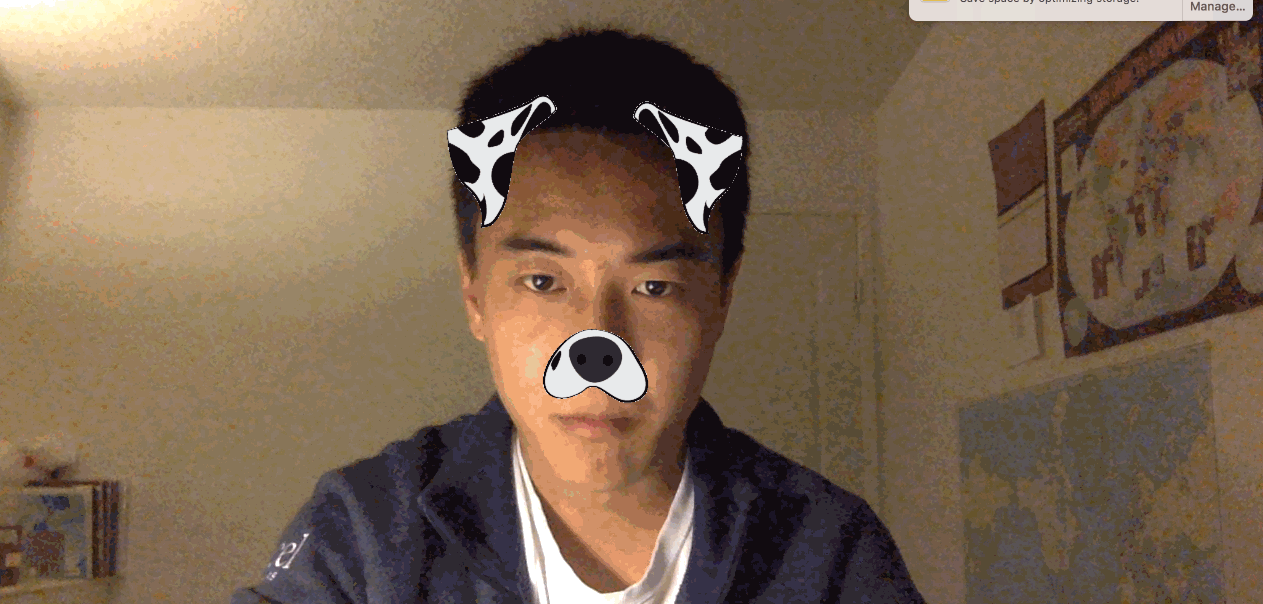As you work through the tutorial, you’ll use `OpenCV`, a computer-vision library, `numpy` for linear algebra utilities, and `matplotlib` for plotting. You’ll also apply the following concepts as you build a computer-vision application:

• Ordinary least squares as a regression and classification technique.
• The basics of stochastic gradient neural networks.

While not necessary to complete this tutorial, you’ll find it easier to understand some of the more detailed explanations if you’re familiar with these mathematical concepts:

• Fundamental linear algebra concepts: scalars, vectors, and matrices.
• Fundamental calculus: how to take a derivative.

You can find the complete code for this tutorial at https://github.com/do-community/emotion-based-dog-filter.

Let’s get started.

## Prerequisites

To complete this tutorial, you will need the following:

## Step 1 — Creating The Project and Installing Dependencies

Let’s create a workspace for this project and install the dependencies we’ll need. We’ll call our workspace `DogFilter`:

``mkdir ~/DogFilter ``

Navigate to the `DogFilter` directory:

``cd ~/DogFilter ``

Then create a new Python virtual environment for the project:

``python3 -m venv dogfilter ``

``source dogfilter/bin/activate ``

The prompt changes, indicating the environment is active. Now install PyTorch, a deep-learning framework for Python that we’ll use in this tutorial. The installation process depends on which operating system you’re using.

On macOS, install Pytorch with the following command:

``python -m pip install torch==0.4.1 torchvision==0.2.1 ``

On Linux, use the following commands:

``pip install http://download.pytorch.org/whl/cpu/torch-0.4.1-cp35-cp35m-linux_x86_64.whl  pip install torchvision ``

And for Windows, install Pytorch with these commands:

``pip install http://download.pytorch.org/whl/cpu/torch-0.4.1-cp35-cp35m-win_amd64.whl  pip install torchvision ``

Now install prepackaged binaries for `OpenCV` and `numpy`, which are computer vision and linear algebra libraries, respectively. The former offers utilities such as image rotations, and the latter offers linear algebra utilities such as a matrix inversion.

``python -m pip install opencv-python==3.4.3.18 numpy==1.14.5 ``

Finally, create a directory for our assets, which will hold the images we’ll use in this tutorial:

``mkdir assets ``

With the dependencies installed, let’s build the first version of our filter: a face detector.

## Step 2 — Building a Face Detector

Our first objective is to detect all faces in an image. We’ll create a script that accepts a single image and outputs an annotated image with the faces outlined with boxes.

Fortunately, instead of writing our own face detection logic, we can use pre-trained models. We’ll set up a model and then load pre-trained parameters. OpenCV makes this easy by providing both.

OpenCV provides the model parameters in its source code. but we need the absolute path to our locally-installed OpenCV to use these parameters. Since that absolute path may vary, we’ll download our own copy instead and place it in the `assets` folder:

``wget -O assets/haarcascade_frontalface_default.xml https://github.com/opencv/opencv/raw/master/data/haarcascades/haarcascade_frontalface_default.xml ``

The `-O` option specifies the destination as `assets/haarcascade_frontalface_default.xml`. The second argument is the source URL.

We’ll detect all faces in the following image from Pexels (CC0, link to original image).First, download the image. The following command saves the downloaded image as `children.png` in the `assets` folder:

``wget -O assets/children.png https://assets.digitalocean.com/articles/python3_dogfilter/CfoBWbF.png ``

To check that the detection algorithm works, we will run it on an individual image and save the resulting annotated image to disk. Create an `outputs` folder for these annotated results.

``mkdir outputs ``

Now create a Python script for the face detector. Create the file `step_1_face_detect` using `nano` or your favorite text editor:

``nano step_2_face_detect.py ``

Add the following code to the file. This code imports OpenCV, which contains the image utilities and face classifier. The rest of the code is typical Python program boilerplate.

step_2_face_detect.py
``"""Test for face detection"""  import cv2   def main():     pass  if __name__ == '__main__':     main() ``

Now replace `pass` in the `main` function with this code which initializes a face classifier using the OpenCV parameters you downloaded to your `assets` folder:

step_2_face_detect.py
``def main():     # initialize front face classifier     cascade = cv2.CascadeClassifier("assets/haarcascade_frontalface_default.xml") ``

Next, add this line to load the image `children.png`.

step_2_face_detect.py
``    frame = cv2.imread('assets/children.png') ``

Then add this code to convert the image to black and white, as the classifier was trained on black-and-white images. To accomplish this, we convert to grayscale and then discretize the histogram:

step_2_face_detect.py
``    # Convert to black-and-white     gray = cv2.cvtColor(frame, cv2.COLOR_BGR2GRAY)     blackwhite = cv2.equalizeHist(gray) ``

Then use OpenCV’s `detectMultiScale` function to detect all faces in the image.

step_2_face_detect.py
``    rects = cascade.detectMultiScale(         blackwhite, scaleFactor=1.3, minNeighbors=4, minSize=(30, 30),         flags=cv2.CASCADE_SCALE_IMAGE) ``
• `scaleFactor` specifies how much the image is reduced along each dimension.
• `minNeighbors` denotes how many neighboring rectangles a candidate rectangle needs to be retained.
• `minSize` is the minimum allowable detected object size. Objects smaller than this are discarded.

The return type is a list of tuples, where each tuple has four numbers denoting the minimum x, minimum y, width, and height of the rectangle in that order.

Iterate over all detected objects and draw them on the image in green using `cv2.rectangle`:

step_2_face_detect.py
``    for x, y, w, h in rects:         cv2.rectangle(frame, (x, y), (x + w, y + h), (0, 255, 0), 2) ``
• The second and third arguments are opposing corners of the rectangle.
• The fourth argument is the color to use. `(0, 255, 0)` corresponds to green for our RGB color space.
• The last argument denotes the width of our line.

Finally, write the image with bounding boxes into a new file at `outputs/children_detected.png`:

step_2_face_detect.py
``    cv2.imwrite('outputs/children_detected.png', frame) ``

Your completed script should look like this:

step_2_face_detect.py
``"""Tests face detection for a static image."""    import cv2     def main():        # initialize front face classifier       cascade = cv2.CascadeClassifier(           "assets/haarcascade_frontalface_default.xml")        frame = cv2.imread('assets/children.png')        # Convert to black-and-white       gray = cv2.cvtColor(frame, cv2.COLOR_BGR2GRAY)       blackwhite = cv2.equalizeHist(gray)        rects = cascade.detectMultiScale(           blackwhite, scaleFactor=1.3, minNeighbors=4, minSize=(30, 30),       flags=cv2.CASCADE_SCALE_IMAGE)        for x, y, w, h in rects:           cv2.rectangle(frame, (x, y), (x + w, y + h), (0, 255, 0), 2)        cv2.imwrite('outputs/children_detected.png', frame)    if __name__ == '__main__':       main() ``

Save the file and exit your editor. Then run the script:

``python step_2_face_detect.py ``

Open `outputs/children_detected.png`. You’ll see the following image that shows the faces outlined with boxes: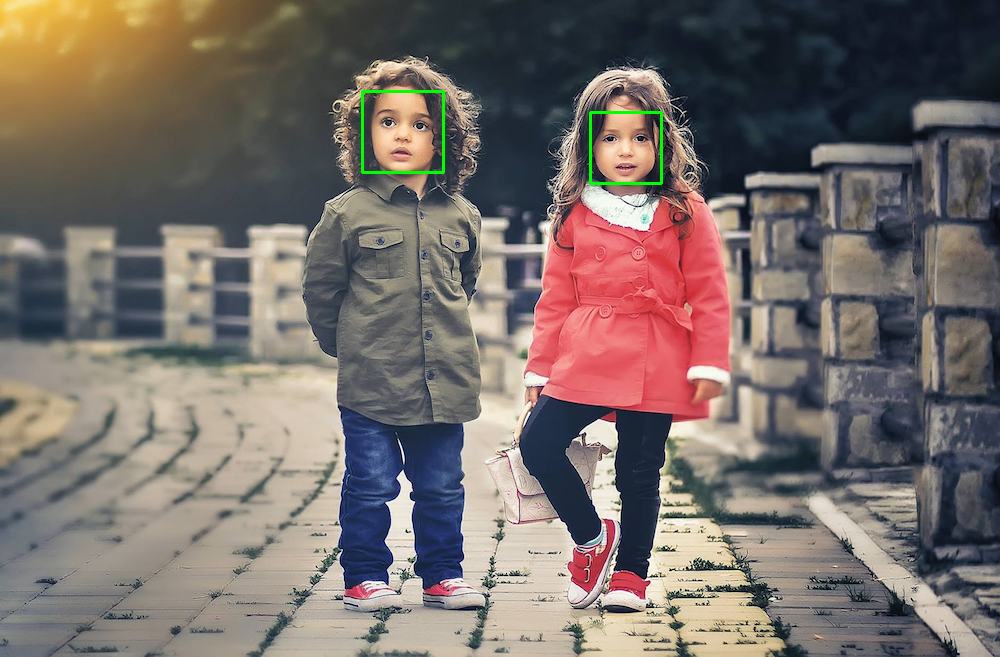At this point, you have a working face detector. It accepts an image as input and draws bounding boxes around all faces in the image, outputting the annotated image. Now let’s apply this same detection to a live camera feed.

## Step 3 — Linking the Camera Feed

The next objective is to link the computer’s camera to the face detector. Instead of detecting faces in a static image, you’ll detect all faces from your computer’s camera. You will collect camera input, detect and annotate all faces, and then display the annotated image back to the user. You’ll continue from the script in Step 2, so start by duplicating that script:

``cp step_2_face_detect.py step_3_camera_face_detect.py ``

Then open the new script in your editor:

``nano step_3_camera_face_detect.py ``

You will update the `main` function by using some elements from this test script from the official OpenCV documentation. Start by initializing a `VideoCapture` object that is set to capture live feed from your computer’s camera. Place this at the start of the `main` function, before the other code in the function:

step_3_camera_face_detect.py
``def main():     cap = cv2.VideoCapture(0)     ... ``

Starting from the line defining `frame`, indent all of your existing code, placing all of the code in a `while` loop.

step_3_camera_face_detect.py
``    while True:         frame = cv2.imread('assets/children.png')         ...         for x, y, w, h in rects:               cv2.rectangle(frame, (x, y), (x + w, y + h), (0, 255, 0), 2)            cv2.imwrite('outputs/children_detected.png', frame) ``

Replace the line defining `frame` at the start of the `while` loop. Instead of reading from an image on disk, you’re now reading from the camera:

step_3_camera_face_detect.py
``    while True:         # frame = cv2.imread('assets/children.png') # DELETE ME         # Capture frame-by-frame         ret, frame = cap.read() ``

Replace the line `cv2.imwrite(...)` at the end of the `while` loop. Instead of writing an image to disk, you’ll display the annotated image back to the user’s screen:

step_3_camera_face_detect.py
``      cv2.imwrite('outputs/children_detected.png', frame)  # DELETE ME       # Display the resulting frame       cv2.imshow('frame', frame) ``

Also, add some code to watch for keyboard input so you can stop the program. Check if the user hits the `q` character and, if so, quit the application. Right after `cv2.imshow(...)` add the following:

step_3_camera_face_detect.py
``...         cv2.imshow('frame', frame)         if cv2.waitKey(1) & 0xFF == ord('q'):             break ... ``

The line `cv2.waitkey(1)` halts the program for 1 millisecond so that the captured image can be displayed back to the user.

Finally, release the capture and close all windows. Place this outside of the `while` loop to end the `main` function.

step_3_camera_face_detect.py
``...      while True:     ...       cap.release()     cv2.destroyAllWindows() ``

Your script should look like the following:

step_3_camera_face_detect.py
``"""Test for face detection on video camera.  Move your face around and a green box will identify your face. With the test frame in focus, hit `q` to exit. Note that typing `q` into your terminal will do nothing. """  import cv2   def main():     cap = cv2.VideoCapture(0)      # initialize front face classifier     cascade = cv2.CascadeClassifier(         "assets/haarcascade_frontalface_default.xml")      while True:         # Capture frame-by-frame         ret, frame = cap.read()          # Convert to black-and-white         gray = cv2.cvtColor(frame, cv2.COLOR_BGR2GRAY)         blackwhite = cv2.equalizeHist(gray)          # Detect faces         rects = cascade.detectMultiScale(             blackwhite, scaleFactor=1.3, minNeighbors=4, minSize=(30, 30),             flags=cv2.CASCADE_SCALE_IMAGE)          # Add all bounding boxes to the image         for x, y, w, h in rects:             cv2.rectangle(frame, (x, y), (x + w, y + h), (0, 255, 0), 2)          # Display the resulting frame         cv2.imshow('frame', frame)         if cv2.waitKey(1) & 0xFF == ord('q'):             break      # When everything done, release the capture     cap.release()     cv2.destroyAllWindows()   if __name__ == '__main__':     main() ``

Save the file and exit your editor.

Now run the test script.

``python step_3_camera_face_detect.py ``

This activates your camera and opens a window displaying your camera’s feed. Your face will be boxed by a green square in real time: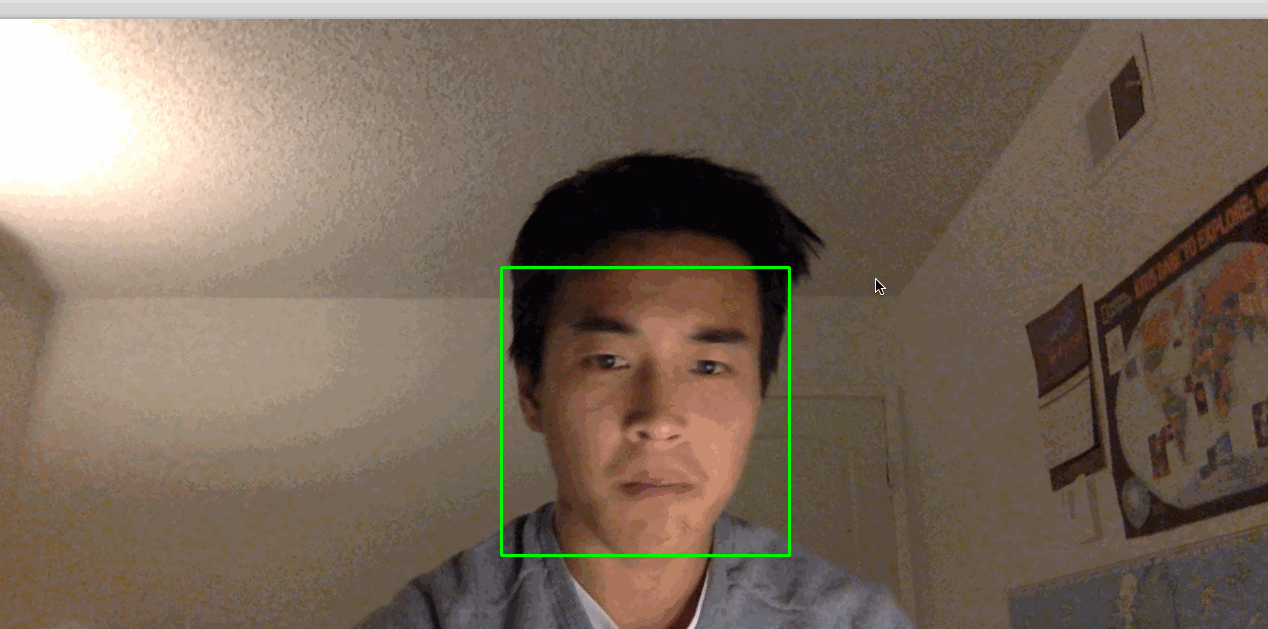Note: If you find that you have to hold very still for things to work, the lighting in the room may not be adequate. Try moving to a brightly lit room where you and your background have high constrast. Also, avoid bright lights near your head. For example, if you have your back to the sun, this process might not work very well.

Our next objective is to take the detected faces and superimpose dog masks on each one.

## Step 4 — Building the Dog Filter

Before we build the filter itself, let’s explore how images are represented numerically. This will give you the background needed to modify images and ultimately apply a dog filter.

Let’s look at an example. We can construct a black-and-white image using numbers, where `0` corresponds to black and `1` corresponds to white.

Focus on the dividing line between 1s and 0s. What shape do you see?

``0 0 0 0 0 0 0 0 0 0 0 0 0 1 0 0 0 0 0 0 0 1 1 1 0 0 0 0 0 1 1 1 1 1 0 0 0 0 0 1 1 1 0 0 0 0 0 0 0 1 0 0 0 0 0 0 0 0 0 0 0 0 0 ``

The image is a diamond. If save this matrix of values as an image. This gives us the following picture:We can use any value between 0 and 1, such as 0.1, 0.26, or 0.74391. Numbers closer to 0 are darker and numbers closer to 1 are lighter. This allows us to represent white, black, and any shade of gray. This is great news for us because we can now construct any grayscale image using 0, 1, and any value in between. Consider the following, for example. Can you tell what it is? Again, each number corresponds to the color of a pixel.

``1  1  1  1  1  1  1  1  1  1  1  1 1  1  1  1  0  0  0  0  1  1  1  1 1  1  0  0 .4 .4 .4 .4  0  0  1  1 1  0 .4 .4 .5 .4 .4 .4 .4 .4  0  1 1  0 .4 .5 .5 .5 .4 .4 .4 .4  0  1 0 .4 .4 .4 .5 .4 .4 .4 .4 .4 .4  0 0 .4 .4 .4 .4  0  0 .4 .4 .4 .4  0 0  0 .4 .4  0  1 .7  0 .4 .4  0  0 0  1  0  0  0 .7 .7  0  0  0  1  0 1  0  1  1  1  0  0 .7 .7 .4  0  1 1  0 .7  1  1  1 .7 .7 .7 .7  0  1 1  1  0  0 .7 .7 .7 .7  0  0  1  1 1  1  1  1  0  0  0  0  1  1  1  1 1  1  1  1  1  1  1  1  1  1  1  1 ``

Re-rendered as an image, you can now tell that this is, in fact, a Poké Ball:You’ve now seen how black-and-white and grayscale images are represented numerically. To introduce color, we need a way to encode more information. An image has its height and width expressed as `h x w`.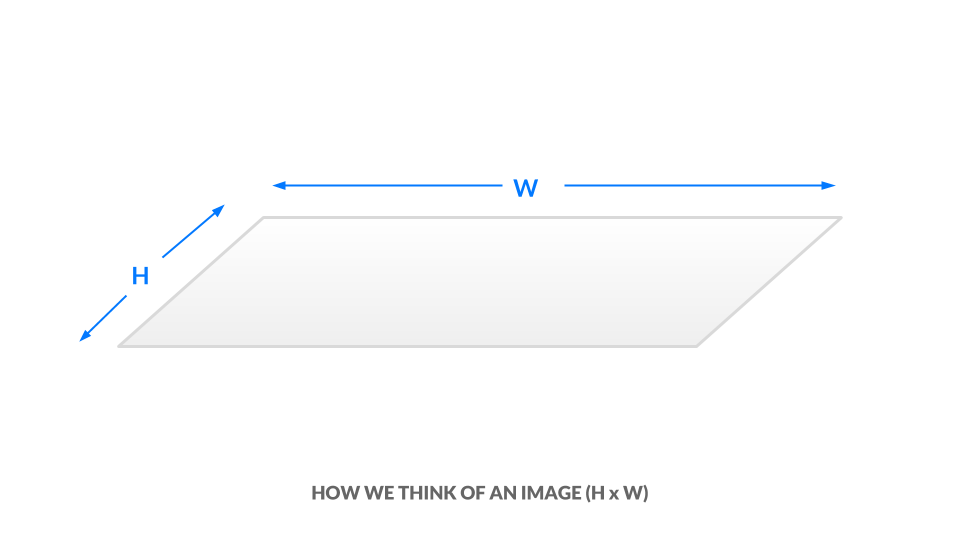In the current grayscale representation, each pixel is one value between 0 and 1. We can equivalently say our image has dimensions `h x w x 1`. In other words, every `(x, y)` position in our image has just one value.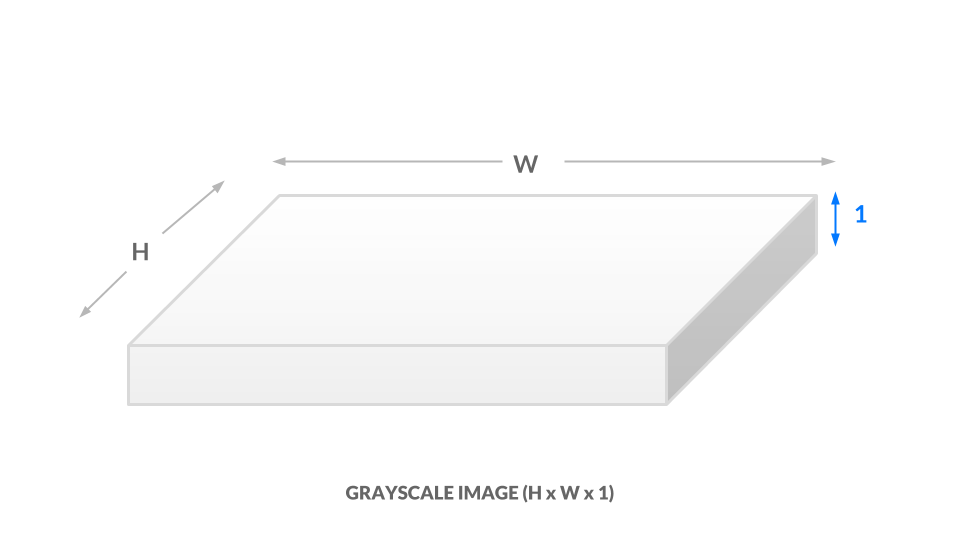For a color representation, we represent the color of each pixel using three values between 0 and 1. One number corresponds to the “degree of red,” one to the “degree of green,” and the last to the “degree of blue.” We call this the RGB color space. This means that for every `(x, y)` position in our image, we have three values `(r, g, b)`. As a result, our image is now `h x w x 3`: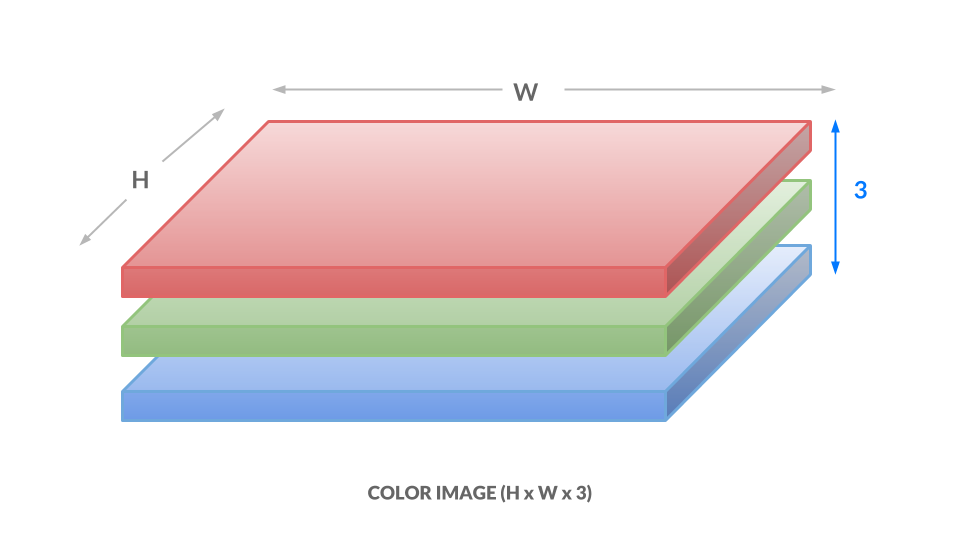Here, each number ranges from 0 to 255 instead of 0 to 1, but the idea is the same. Different combinations of numbers correspond to different colors, such as dark purple `(102, 0, 204)` or bright orange `(255, 153, 51)`. The takeaways are as follows:

1. Each image will be represented as a box of numbers that has three dimensions: height, width, and color channels. Manipulating this box of numbers directly is equivalent to manipulating the image.
2. We can also flatten this box to become just a list of numbers. In this way, our image becomes a vector. Later on, we will refer to images as vectors.

Now that you understand how images are represented numerically, you are well-equipped to begin applying dog masks to faces. To apply a dog mask, you will replace values in the child image with non-white dog mask pixels. To start, you will work with a single image. Download this crop of a face from the image you used in Step 2.

``wget -O assets/child.png https://assets.digitalocean.com/articles/python3_dogfilter/alXjNK1.png ``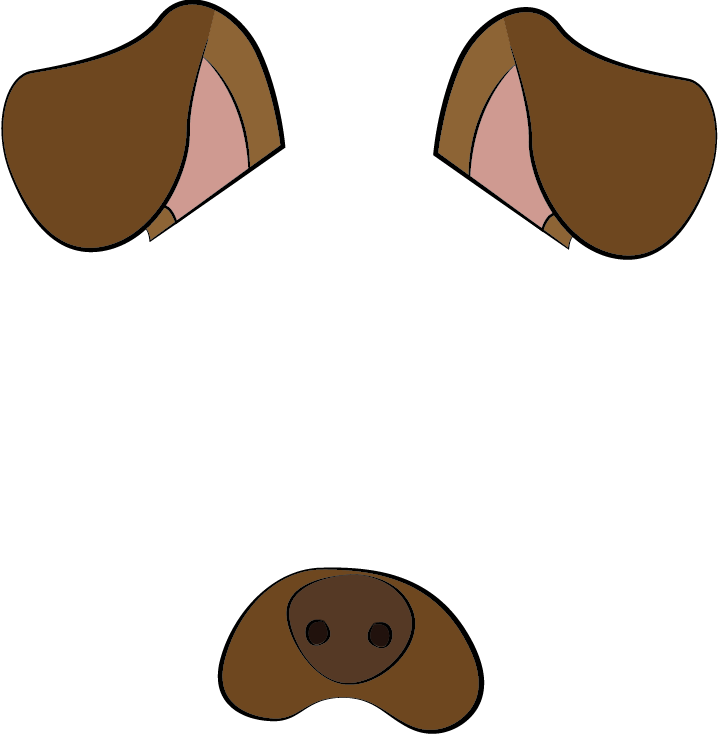Download this with `wget`:

``wget -O assets/dog.png https://assets.digitalocean.com/articles/python3_dogfilter/ED32BCs.png ``

Create a new file called `step_4_dog_mask_simple.py` which will hold the code for the script that applies the dog mask to faces:

``nano step_4_dog_mask_simple.py ``

Add the following boilerplate for the Python script and import the OpenCV and `numpy` libraries:

``"""Test for adding dog mask"""  import cv2 import numpy as np   def main():     pass  if __name__ == '__main__':     main() ``

Replace `pass` in the `main` function with these two lines which load the original image and the dog mask into memory.

``... def main():     face = cv2.imread('assets/child.png')     mask = cv2.imread('assets/dog.png') ``

Next, fit the dog mask to the child. The logic is more complicated than what we’ve done previously, so we will create a new function called `apply_mask` to modularize our code. Directly after the two lines that load the images, add this line which invokes the `apply_mask` function:

``...     face_with_mask = apply_mask(face, mask) ``

Create a new function called `apply_mask` and place it above the `main` function:

``... def apply_mask(face: np.array, mask: np.array) -> np.array:     """Add the mask to the provided face, and return the face with mask."""     pass  def main(): ... ``

At this point, your file should look like this:

``"""Test for adding dog mask"""  import cv2 import numpy as np   def apply_mask(face: np.array, mask: np.array) -> np.array:     """Add the mask to the provided face, and return the face with mask."""     pass   def main():     face = cv2.imread('assets/child.png')     mask = cv2.imread('assets/dog.png')     face_with_mask = apply_mask(face, mask)  if __name__ == '__main__':     main() ``

Let’s build out the `apply_mask` function. Our goal is to apply the mask to the child’s face. However, we need to maintain the aspect ratio for our dog mask. To do so, we need to explicitly compute our dog mask’s final dimensions. Inside the `apply_mask` function, replace `pass` with these two lines which extract the height and width of both images:

``...     mask_h, mask_w, _ = mask.shape     face_h, face_w, _ = face.shape ``

Next, determine which dimension needs to be “shrunk more.” To be precise, we need the tighter of the two constraints. Add this line to the `apply_mask` function:

``...      # Resize the mask to fit on face     factor = min(face_h / mask_h, face_w / mask_w) ``

Then compute the new shape by adding this code to the function:

``...     new_mask_w = int(factor * mask_w)     new_mask_h = int(factor * mask_h)     new_mask_shape = (new_mask_w, new_mask_h) ``

Here we cast the numbers to integers, as the `resize` function needs integral dimensions.

Now add this code to resize the dog mask to the new shape:

``...      # Add mask to face - ensure mask is centered     resized_mask = cv2.resize(mask, new_mask_shape) ``

Finally, write the image to disk so you can double-check that your resized dog mask is correct after you run the script:

``    cv2.imwrite('outputs/resized_dog.png', resized_mask) ``

The completed script should look like this:

``"""Test for adding dog mask""" import cv2 import numpy as np  def apply_mask(face: np.array, mask: np.array) -> np.array:     """Add the mask to the provided face, and return the face with mask."""     mask_h, mask_w, _ = mask.shape     face_h, face_w, _ = face.shape      # Resize the mask to fit on face     factor = min(face_h / mask_h, face_w / mask_w)     new_mask_w = int(factor * mask_w)     new_mask_h = int(factor * mask_h)     new_mask_shape = (new_mask_w, new_mask_h)      # Add mask to face - ensure mask is centered     resized_mask = cv2.resize(mask, new_mask_shape)     cv2.imwrite('outputs/resized_dog.png', resized_mask)   def main():     face = cv2.imread('assets/child.png')     mask = cv2.imread('assets/dog.png')     face_with_mask = apply_mask(face, mask)  if __name__ == '__main__':     main()  ``

Save the file and exit your editor. Run the new script:

``python step_4_dog_mask_simple.py ``

Open the image at `outputs/resized_dog.png` to double-check the mask was resized correctly. It will match the dog mask shown earlier in this section.

Now add the dog mask to the child. Open the `step_4_dog_mask_simple.py` file again and return to the `apply_mask` function:

``nano step_4_dog_mask_simple.py ``

First, remove the line of code that writes the resized mask from the `apply_mask` function since you no longer need it:

``    cv2.imwrite('outputs/resized_dog.png', resized_mask)  # delete this line     ... ``

In its place, apply your knowledge of image representation from the start of this section to modify the image. Start by making a copy of the child image. Add this line to the `apply_mask` function:

``...     face_with_mask = face.copy() ``

Next, find all positions where the dog mask is not white or near white. To do this, check if the pixel value is less than 250 across all color channels, as we’d expect a near-white pixel to be near `[255, 255, 255]`. Add this code:

``...     non_white_pixels = (resized_mask < 250).all(axis=2) ``

At this point, the dog image is, at most, as large as the child image. We want to center the dog image on the face, so compute the offset needed to center the dog image by adding this code to `apply_mask`:

``...     off_h = int((face_h - new_mask_h) / 2)       off_w = int((face_w - new_mask_w) / 2) ``

Copy all non-white pixels from the dog image into the child image. Since the child image may be larger than the dog image, we need to take a subset of the child image:

``    face_with_mask[off_h: off_h+new_mask_h, off_w: off_w+new_mask_w][non_white_pixels] = \             resized_mask[non_white_pixels] ``

Then return the result:

``    return face_with_mask ``

In the `main` function, add this code to write the result of the `apply_mask` function to an output image so you can manually double-check the result:

``...     face_with_mask = apply_mask(face, mask)     cv2.imwrite('outputs/child_with_dog_mask.png', face_with_mask) ``

Your completed script will look like the following:

``"""Test for adding dog mask"""  import cv2 import numpy as np   def apply_mask(face: np.array, mask: np.array) -> np.array:     """Add the mask to the provided face, and return the face with mask."""     mask_h, mask_w, _ = mask.shape     face_h, face_w, _ = face.shape      # Resize the mask to fit on face     factor = min(face_h / mask_h, face_w / mask_w)     new_mask_w = int(factor * mask_w)     new_mask_h = int(factor * mask_h)     new_mask_shape = (new_mask_w, new_mask_h)     resized_mask = cv2.resize(mask, new_mask_shape)      # Add mask to face - ensure mask is centered     face_with_mask = face.copy()     non_white_pixels = (resized_mask < 250).all(axis=2)     off_h = int((face_h - new_mask_h) / 2)       off_w = int((face_w - new_mask_w) / 2)     face_with_mask[off_h: off_h+new_mask_h, off_w: off_w+new_mask_w][non_white_pixels] = \          resized_mask[non_white_pixels]      return face_with_mask  def main():     face = cv2.imread('assets/child.png')     mask = cv2.imread('assets/dog.png')     face_with_mask = apply_mask(face, mask)     cv2.imwrite('outputs/child_with_dog_mask.png', face_with_mask)  if __name__ == '__main__':     main() ``

Save the script and run it:

``python step_4_dog_mask_simple.py ``

You’ll have the following picture of a child with a dog mask in `outputs/child_with_dog_mask.png`: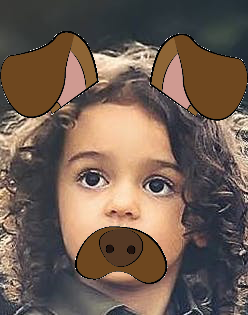You now have a utility that applies dog masks to faces. Now let’s use what you’ve built to add the dog mask in real time.

We’ll pick up from where we left off in Step 3. Copy `step_3_camera_face_detect.py` to `step_4_dog_mask.py`.

``cp step_3_camera_face_detect.py step_4_dog_mask.py ``

``nano step_4_dog_mask.py ``

First, import the NumPy library at the top of the script:

``import numpy as np ... ``

Then add the `apply_mask` function from your previous work into this new file above the `main` function:

``def apply_mask(face: np.array, mask: np.array) -> np.array:     """Add the mask to the provided face, and return the face with mask."""     mask_h, mask_w, _ = mask.shape     face_h, face_w, _ = face.shape      # Resize the mask to fit on face     factor = min(face_h / mask_h, face_w / mask_w)     new_mask_w = int(factor * mask_w)     new_mask_h = int(factor * mask_h)     new_mask_shape = (new_mask_w, new_mask_h)     resized_mask = cv2.resize(mask, new_mask_shape)      # Add mask to face - ensure mask is centered     face_with_mask = face.copy()     non_white_pixels = (resized_mask < 250).all(axis=2)     off_h = int((face_h - new_mask_h) / 2)       off_w = int((face_w - new_mask_w) / 2)     face_with_mask[off_h: off_h+new_mask_h, off_w: off_w+new_mask_w][non_white_pixels] = \          resized_mask[non_white_pixels]      return face_with_mask ... ``

Second, locate this line in the `main` function:

``    cap = cv2.VideoCapture(0) ``

``    cap = cv2.VideoCapture(0)      # load mask     mask = cv2.imread('assets/dog.png')     ... ``

Next, in the `while` loop, locate this line:

``        ret, frame = cap.read() ``

Add this line after it to extract the image’s height and width:

``        ret, frame = cap.read()         frame_h, frame_w, _ = frame.shape         ... ``

Next, delete the line in `main` that draws bounding boxes. You’ll find this line in the `for` loop that iterates over detected faces:

``        for x, y, w, h in rects:         ...             cv2.rectangle(frame, (x, y), (x + w, y + h), (0, 255, 0), 2) # DELETE ME         ... ``

In its place, add this code which crops the frame. For aesthetic purposes, we crop an area slightly larger than the face.

``        for x, y, w, h in rects:             # crop a frame slightly larger than the face             y0, y1 = int(y - 0.25*h), int(y + 0.75*h)             x0, x1 = x, x + w ``

Introduce a check in case the detected face is too close to the edge.

``            # give up if the cropped frame would be out-of-bounds             if x0 < 0 or y0 < 0 or x1 > frame_w or y1 > frame_h:                 continue ``

Finally, insert the face with a mask into the image.

``            # apply mask             frame[y0: y1, x0: x1] = apply_mask(frame[y0: y1, x0: x1], mask) ``

Verify that your script looks like this:

``"""Real-time dog filter  Move your face around and a dog filter will be applied to your face if it is not out-of-bounds. With the test frame in focus, hit `q` to exit. Note that typing `q` into your terminal will do nothing. """  import numpy as np import cv2   def apply_mask(face: np.array, mask: np.array) -> np.array:     """Add the mask to the provided face, and return the face with mask."""     mask_h, mask_w, _ = mask.shape     face_h, face_w, _ = face.shape      # Resize the mask to fit on face     factor = min(face_h / mask_h, face_w / mask_w)     new_mask_w = int(factor * mask_w)     new_mask_h = int(factor * mask_h)     new_mask_shape = (new_mask_w, new_mask_h)     resized_mask = cv2.resize(mask, new_mask_shape)      # Add mask to face - ensure mask is centered     face_with_mask = face.copy()     non_white_pixels = (resized_mask < 250).all(axis=2)     off_h = int((face_h - new_mask_h) / 2)     off_w = int((face_w - new_mask_w) / 2)     face_with_mask[off_h: off_h+new_mask_h, off_w: off_w+new_mask_w][non_white_pixels] = \          resized_mask[non_white_pixels]      return face_with_mask  def main():     cap = cv2.VideoCapture(0)      # load mask     mask = cv2.imread('assets/dog.png')      # initialize front face classifier     cascade = cv2.CascadeClassifier("assets/haarcascade_frontalface_default.xml")      while(True):         # Capture frame-by-frame         ret, frame = cap.read()         frame_h, frame_w, _ = frame.shape          # Convert to black-and-white         gray = cv2.cvtColor(frame, cv2.COLOR_BGR2GRAY)         blackwhite = cv2.equalizeHist(gray)          # Detect faces         rects = cascade.detectMultiScale(             blackwhite, scaleFactor=1.3, minNeighbors=4, minSize=(30, 30),             flags=cv2.CASCADE_SCALE_IMAGE)          # Add mask to faces         for x, y, w, h in rects:             # crop a frame slightly larger than the face             y0, y1 = int(y - 0.25*h), int(y + 0.75*h)             x0, x1 = x, x + w              # give up if the cropped frame would be out-of-bounds             if x0 < 0 or y0 < 0 or x1 > frame_w or y1 > frame_h:                 continue              # apply mask             frame[y0: y1, x0: x1] = apply_mask(frame[y0: y1, x0: x1], mask)          # Display the resulting frame         cv2.imshow('frame', frame)         if cv2.waitKey(1) & 0xFF == ord('q'):             break      # When everything done, release the capture     cap.release()     cv2.destroyAllWindows()   if __name__ == '__main__':     main() ``

Save the file and exit your editor. Then run the script.

``python step_4_dog_mask.py ``

You now have a real-time dog filter running. The script will also work with multiple faces in the picture, so you can get your friends together for some automatic dog-ification.This concludes our first primary objective in this tutorial, which is to create a Snapchat-esque dog filter. Now let’s use facial expression to determine the dog mask applied to a face.

## Step 5 — Build a Basic Face Emotion Classifier using Least Squares

In this section you’ll create an emotion classifier to apply different masks based on displayed emotions. If you smile, the filter will apply a corgi mask. If you frown, it will apply a pug mask. Along the way, you’ll explore the least-squares framework, which is fundamental to understanding and discussing machine learning concepts.

To understand how to process our data and produce predictions, we’ll first briefly explore machine learning models.

We need to ask two questions for each model that we consider. For now, these two questions will be sufficient to differentiate between models:

1. Input: What information is the model given?
2. Output: What is the model trying to predict?

At a high-level, the goal is to develop a model for emotion classification. The model is:

1. Input: given images of faces.
2. Output: predicts the corresponding emotion.
``model: face -> emotion ``

The approach we’ll use is least squares; we take a set of points, and we find a line of best fit. The line of best fit, shown in the following image, is our model.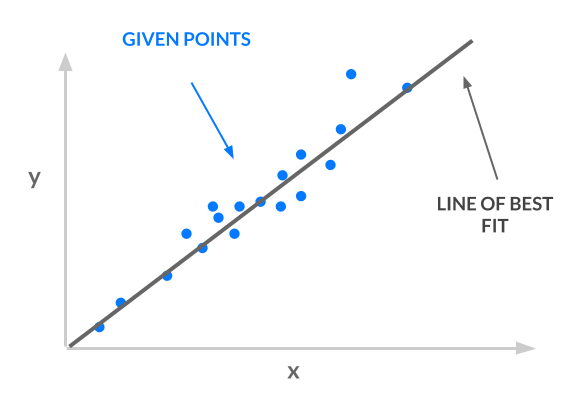Consider the input and output for our line:

1. Input: given `x` coordinates.
2. Output: predicts the corresponding \$ y\$ coordinate.
``least squares line: x -> y ``

Our input `x` must represent faces and our output `y` must represent emotion, in order for us to use least squares for emotion classification:

• `x -> face`: Instead of using one number for `x`, we will use a vector of values for `x`. Thus, `x` can represent images of faces. The article Ordinary Least Squares explains why you can use a vector of values for `x`.
• `y -> emotion`: Each emotion will correspond to a number. For example, “angry” is 0, “sad” is 1, and “happy” is 2. In this way, `y` can represent emotions. However, our line is not constrained to output the `y` values 0, 1, and 2. It has an infinite number of possible y values–it could be 1.2, 3.5, or 10003.42. How do we translate those `y` values to integers corresponding to classes? See the article One-Hot Encoding for more detail and explanation.

Armed with this background knowledge, you will build a simple least-squares classifier using vectorized images and one-hot encoded labels. You’ll accomplish this in three steps:

1. Preprocess the data: As explained at the start of this section, our samples are vectors where each vector encodes an image of a face. Our labels are integers corresponding to an emotion, and we’ll apply one-hot encoding to these labels.
2. Specify and train the model: Use the closed-form least squares solution, `w^*`.
3. Run a prediction using the model: Take the argmax of `Xw^*` to obtain predicted emotions.

Let’s get started.

First, set up a directory to contain the data:

``mkdir data ``

Then download the data, curated by Pierre-Luc Carrier and Aaron Courville, from a 2013 Face Emotion Classification competition on Kaggle.

``wget -O data/fer2013.tar https://bitbucket.org/alvinwan/adversarial-examples-in-computer-vision-building-then-fooling/raw/babfe4651f89a398c4b3fdbdd6d7a697c5104cff/fer2013.tar ``

Navigate to the `data` directory and unpack the data.

``cd data tar -xzf fer2013.tar ``

Now we’ll create a script to run the least-squares model. Navigate to the root of your project:

``cd ~/DogFilter ``

Create a new file for the script:

``nano step_5_ls_simple.py ``

Add Python boilerplate and import the packages you will need:

step_5_ls_simple.py
``"""Train emotion classifier using least squares."""  import numpy as np  def main():     pass  if __name__ == '__main__':     main() ``

Next, load the data into memory. Replace `pass` in your `main` function with the following code:

step_5_ls_simple.py
``     # load data     with np.load('data/fer2013_train.npz') as data:         X_train, Y_train = data['X'], data['Y']      with np.load('data/fer2013_test.npz') as data:         X_test, Y_test = data['X'], data['Y'] ``

Now one-hot encode the labels. To do this, construct the identity matrix with `numpy` and then index into this matrix using our list of labels:

step_5_ls_simple.py
``    # one-hot labels     I = np.eye(6)     Y_oh_train, Y_oh_test = I[Y_train], I[Y_test] ``

Here, we use the fact that the `i`-th row in the identity matrix is all zero, except for the `i`-th entry. Thus, the i-th row is the one-hot encoding for the label of class `i`. Additionally, we use `numpy`‘s advanced indexing, where `[a, b, c, d][[1, 3]] = [b, d]`.

Computing `(X^TX)^{-1}` would take too long on commodity hardware, as `X^TX` is a `2304x2304` matrix with over four million values, so we’ll reduce this time by selecting only the first 100 features. Add this code:

step_5_ls_simple.py
``...     # select first 100 dimensions     A_train, A_test = X_train[:, :100], X_test[:, :100] ``

Next, add this code to evaluate the closed-form least-squares solution:

step_5_ls_simple.py
``...     # train model     w = np.linalg.inv(A_train.T.dot(A_train)).dot(A_train.T.dot(Y_oh_train)) ``

Then define an evaluation function for training and validation sets. Place this before your `main` function:

step_5_ls_simple.py
``def evaluate(A, Y, w):     Yhat = np.argmax(A.dot(w), axis=1)     return np.sum(Yhat == Y) / Y.shape ``

To estimate labels, we take the inner product with each sample and get the indices of the maximum values using `np.argmax`. Then we compute the average number of correct classifications. This final number is your accuracy.

Finally, add this code to the end of the `main` function to compute the training and validation accuracy using the `evaluate` function you just wrote:

step_5_ls_simple.py
``    # evaluate model     ols_train_accuracy = evaluate(A_train, Y_train, w)     print('(ols) Train Accuracy:', ols_train_accuracy)     ols_test_accuracy = evaluate(A_test, Y_test, w)     print('(ols) Test Accuracy:', ols_test_accuracy) ``

Double-check that your script matches the following:

step_5_ls_simple.py
``"""Train emotion classifier using least squares."""  import numpy as np   def evaluate(A, Y, w):     Yhat = np.argmax(A.dot(w), axis=1)     return np.sum(Yhat == Y) / Y.shape  def main():      # load data     with np.load('data/fer2013_train.npz') as data:         X_train, Y_train = data['X'], data['Y']      with np.load('data/fer2013_test.npz') as data:         X_test, Y_test = data['X'], data['Y']      # one-hot labels     I = np.eye(6)     Y_oh_train, Y_oh_test = I[Y_train], I[Y_test]      # select first 100 dimensions     A_train, A_test = X_train[:, :100], X_test[:, :100]      # train model     w = np.linalg.inv(A_train.T.dot(A_train)).dot(A_train.T.dot(Y_oh_train))      # evaluate model     ols_train_accuracy = evaluate(A_train, Y_train, w)     print('(ols) Train Accuracy:', ols_train_accuracy)     ols_test_accuracy = evaluate(A_test, Y_test, w)     print('(ols) Test Accuracy:', ols_test_accuracy)   if __name__ == '__main__':     main() ``

``python step_5_ls_simple.py ``

You’ll see the following output:

``Output(ols) Train Accuracy: 0.4748918316507146 (ols) Test Accuracy: 0.45280545359202934 ``

Our model gives 47.5% train accuracy. We repeat this on the validation set to obtain 45.3% accuracy. For a three-way classification problem, 45.3% is reasonably above guessing, which is 33\%​. This is our starting classifier for emotion detection, and in the next step, you’ll build off of this least-squares model to improve accuracy. The higher the accuracy, the more reliably your emotion-based dog filter can find the appropriate dog filter for each detected emotion.

## Step 6 — Improving Accuracy by Featurizing the Inputs

We can use a more expressive model to boost accuracy. To accomplish this, we featurize our inputs.

The original image tells us that position (`0, 0`) is red, (`1, 0`) is brown, and so on. A featurized image may tell us that there is a dog to the top-left of the image, a person in the middle, etc. Featurization is powerful, but its precise definition is beyond the scope of this tutorial.

We’ll use an approximation for the radial basis function (RBF) kernel, using a random Gaussian matrix. We won’t go into detail in this tutorial. Instead, we’ll treat this as a black box that computes higher-order features for us.

We’ll continue where we left off in the previous step. Copy the previous script so you have a good starting point:

``cp step_5_ls_simple.py step_6_ls_simple.py ``

Open the new file in your editor:

``nano step_6_ls_simple.py ``

We’ll start by creating the featurizing random matrix. Again, we’ll use only 100 features in our new feature space.

Locate the following line, defining `A_train` and `A_test`:

step_6_ls_simple.py
``    # select first 100 dimensions     A_train, A_test = X_train[:, :100], X_test[:, :100] ``

Directly above this definition for `A_train` and `A_test`, add a random feature matrix:

step_6_ls_simple.py
``    d = 100     W = np.random.normal(size=(X_train.shape, d))     # select first 100 dimensions     A_train, A_test = X_train[:, :100], X_test[:, :100]  ... ``

Then replace the definitions for `A_train` and `A_test`. We redefine our matrices, called design matrices, using this random featurization.

step_6_ls_simple.py
``    A_train, A_test = X_train.dot(W), X_test.dot(W) ``

Save your file and run the script.

``python step_6_ls_simple.py ``

You’ll see the following output:

``Output(ols) Train Accuracy: 0.584174642717 (ols) Test Accuracy: 0.584425799685 ``

This featurization now offers 58.4% train accuracy and 58.4% validation accuracy, a 13.1% improvement in validation results. We trimmed the X matrix to be `100 x 100`, but the choice of 100 was arbirtary. We could also trim the `X` matrix to be `1000 x 1000` or `50 x 50`. Say the dimension of `x` is `d x d`. We can test more values of `d` by re-trimming X to be `d x d` and recomputing a new model.

Trying more values of `d`, we find an additional 4.3% improvement in test accuracy to 61.7%. In the following figure, we consider the performance of our new classifier as we vary `d`. Intuitively, as `d` increases, the accuracy should also increase, as we use more and more of our original data. Rather than paint a rosy picture, however, the graph exhibits a negative trend: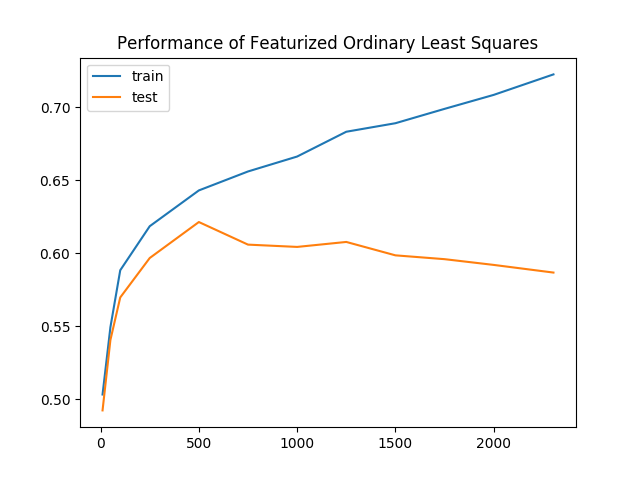As we keep more of our data, the gap between the training and validation accuracies increases as well. This is clear evidence of overfitting, where our model is learning representations that are no longer generalizable to all data. To combat overfitting, we’ll regularize our model by penalizing complex models.

We amend our ordinary least-squares objective function with a regularization term, giving us a new objective. Our new objective function is called ridge regression and it looks like this:

``min_w |Aw- y|^2 + lambda |w|^2 ``

In this equation, `lambda` is a tunable hyperparameter. Plug `lambda = 0` into the equation and ridge regression becomes least-squares. Plug `lambda = infinity` into the equation, and you’ll find the best `w` must now be zero, as any non-zero `w` incurs infinite loss. As it turns out, this objective yields a closed-form solution as well:

``w^* = (A^TA + lambda I)^{-1}A^Ty ``

Still using the featurized samples, retrain and reevaluate the model once more.

Open `step_6_ls_simple.py` again in your editor:

``nano step_6_ls_simple.py ``

This time, increase the dimensionality of the new feature space to `d=1000​`. Change the value of `d` from `100` to `1000` as shown in the following code block:

step_6_ls_simple.py
``...     d = 1000     W = np.random.normal(size=(X_train.shape, d)) ... ``

Then apply ridge regression using a regularization of `lambda = 10^{10}`. Replace the line defining `w` with the following two lines:

step_6_ls_simple.py
``...     # train model     I = np.eye(A_train.shape)     w = np.linalg.inv(A_train.T.dot(A_train) + 1e10 * I).dot(A_train.T.dot(Y_oh_train)) ``

Then locate this block:

step_6_ls_simple.py
``...   ols_train_accuracy = evaluate(A_train, Y_train, w)   print('(ols) Train Accuracy:', ols_train_accuracy)   ols_test_accuracy = evaluate(A_test, Y_test, w)   print('(ols) Test Accuracy:', ols_test_accuracy) ``

Replace it with the following:

step_6_ls_simple.py
``...    print('(ridge) Train Accuracy:', evaluate(A_train, Y_train, w))   print('(ridge) Test Accuracy:', evaluate(A_test, Y_test, w)) ``

The completed script should look like this:

step_6_ls_simple.py
``"""Train emotion classifier using least squares."""  import numpy as np  def evaluate(A, Y, w):     Yhat = np.argmax(A.dot(w), axis=1)     return np.sum(Yhat == Y) / Y.shape  def main():     # load data     with np.load('data/fer2013_train.npz') as data:         X_train, Y_train = data['X'], data['Y']      with np.load('data/fer2013_test.npz') as data:         X_test, Y_test = data['X'], data['Y']      # one-hot labels     I = np.eye(6)     Y_oh_train, Y_oh_test = I[Y_train], I[Y_test]     d = 1000     W = np.random.normal(size=(X_train.shape, d))     # select first 100 dimensions     A_train, A_test = X_train.dot(W), X_test.dot(W)      # train model     I = np.eye(A_train.shape)     w = np.linalg.inv(A_train.T.dot(A_train) + 1e10 * I).dot(A_train.T.dot(Y_oh_train))      # evaluate model     print('(ridge) Train Accuracy:', evaluate(A_train, Y_train, w))     print('(ridge) Test Accuracy:', evaluate(A_test, Y_test, w))  if __name__ == '__main__':     main() ``

Save the file, exit your editor, and run the script:

``python step_6_ls_simple.py ``

You’ll see the following output:

``Output(ridge) Train Accuracy: 0.651173462698 (ridge) Test Accuracy: 0.622181436812 ``

There’s an additional improvement of 0.4% in validation accuracy to 62.2%, as train accuracy drops to 65.1%. Once again reevaluating across a number of different `d`, we see a smaller gap between training and validation accuracies for ridge regression. In other words, ridge regression was subject to less overfitting.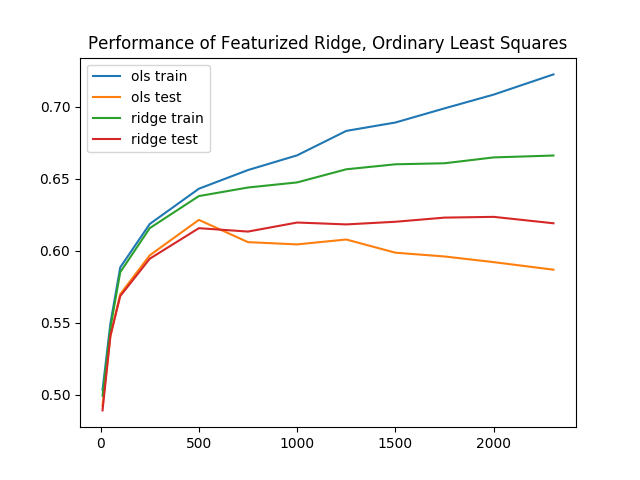Baseline performance for least squares, with these extra enhancements, performs reasonably well. The training and inference times, all together, take no more than 20 seconds for even the best results. In the next section, you’ll explore even more complex models.

## Step 7 — Building the Face-Emotion Classifier Using a Convolutional Neural Network in PyTorch

In this section, you’ll build a second emotion classifier using neural networks instead of least squares. Again, our goal is to produce a model that accepts faces as input and outputs an emotion. Eventually, this classifier will then determine which dog mask to apply.

For a brief neural network visualization and introduction, see the article Understanding Neural Networks. Here, we will use a deep-learning library called PyTorch. There are a number of deep-learning libraries in widespread use, and each has various pros and cons. PyTorch is a particularly good place to start. To impliment this neural network classifier, we again take three steps, as we did with the least-squares classifier:

1. Preprocess the data: Apply one-hot encoding and then apply PyTorch abstractions.
2. Specify and train the model: Set up a neural network using PyTorch layers. Define optimization hyperparameters and run stochastic gradient descent.
3. Run a prediction using the model: Evaluate the neural network.

Create a new file, named `step_7_fer_simple.py`

``nano step_7_fer_simple.py ``

Import the necessary utilities and create a Python class that will hold your data. For data processing here, you will create the train and test datasets. To do these, implement PyTorch’s `Dataset` interface, which lets you load and use PyTorch’s built-in data pipeline for the face-emotion recognition dataset:

step_7_fer_simple.py
``from torch.utils.data import Dataset from torch.autograd import Variable import torch.nn as nn import torch.nn.functional as F import torch.optim as optim import numpy as np import torch import cv2 import argparse   class Fer2013Dataset(Dataset):     """Face Emotion Recognition dataset.      Utility for loading FER into PyTorch. Dataset curated by Pierre-Luc Carrier     and Aaron Courville in 2013.      Each sample is 1 x 1 x 48 x 48, and each label is a scalar.     """     pass ``

Delete the `pass` placeholder in the `Fer2013Dataset` class. In its place, add a function that will initialize our data holder:

step_7_fer_simple.py
``    def __init__(self, path: str):         """         Args:             path: Path to `.np` file containing sample nxd and label nx1         """         with np.load(path) as data:             self._samples = data['X']             self._labels = data['Y']         self._samples = self._samples.reshape((-1, 1, 48, 48))          self.X = Variable(torch.from_numpy(self._samples)).float()         self.Y = Variable(torch.from_numpy(self._labels)).float() ... ``

This function starts by loading the samples and labels. Then it wraps the data in PyTorch data structures.

Directly after the `__init__` function, add a `__len__` function, as this is needed to implement the `Dataset` interface PyTorch expects:

step_7_fer_simple.py
``...     def __len__(self):         return len(self._labels) ``

Finally, add a `__getitem__` method, which returns a dictionary containing the sample and the label:

step_7_fer_simple.py
``    def __getitem__(self, idx):         return {'image': self._samples[idx], 'label': self._labels[idx]} ``

Double-check that your file looks like the following:

step_7_fer_simple.py
``from torch.utils.data import Dataset from torch.autograd import Variable import torch.nn as nn import torch.nn.functional as F import torch.optim as optim import numpy as np import torch import cv2 import argparse   class Fer2013Dataset(Dataset):     """Face Emotion Recognition dataset.     Utility for loading FER into PyTorch. Dataset curated by Pierre-Luc Carrier     and Aaron Courville in 2013.     Each sample is 1 x 1 x 48 x 48, and each label is a scalar.     """      def __init__(self, path: str):         """         Args:             path: Path to `.np` file containing sample nxd and label nx1         """         with np.load(path) as data:             self._samples = data['X']             self._labels = data['Y']         self._samples = self._samples.reshape((-1, 1, 48, 48))          self.X = Variable(torch.from_numpy(self._samples)).float()         self.Y = Variable(torch.from_numpy(self._labels)).float()      def __len__(self):         return len(self._labels)      def __getitem__(self, idx):         return {'image': self._samples[idx], 'label': self._labels[idx]} ``

Next, load the `Fer2013Dataset` dataset. Add the following code to the end of your file after the `Fer2013Dataset` class:

step_7_fer_simple.py
``trainset = Fer2013Dataset('data/fer2013_train.npz') trainloader = torch.utils.data.DataLoader(trainset, batch_size=32, shuffle=True)  testset = Fer2013Dataset('data/fer2013_test.npz') testloader = torch.utils.data.DataLoader(testset, batch_size=32, shuffle=False) ``

This code initializes the dataset using the `Fer2013Dataset` class you created. Then for the train and validation sets, it wraps the dataset in a `DataLoader`. This translates the dataset into an iterable to use later.

As a sanity check, verify that the dataset utilities are functioning. Create a sample dataset loader using `DataLoader` and print the first element of that loader. Add the following to the end of your file:

step_7_fer_simple.py
``if __name__ == '__main__':     loader = torch.utils.data.DataLoader(trainset, batch_size=2, shuffle=False)     print(next(iter(loader))) ``

Verify that your completed script looks like this:

step_7_fer_simple.py
``from torch.utils.data import Dataset from torch.autograd import Variable import torch.nn as nn import torch.nn.functional as F import torch.optim as optim import numpy as np import torch import cv2 import argparse   class Fer2013Dataset(Dataset):     """Face Emotion Recognition dataset.     Utility for loading FER into PyTorch. Dataset curated by Pierre-Luc Carrier     and Aaron Courville in 2013.     Each sample is 1 x 1 x 48 x 48, and each label is a scalar.     """      def __init__(self, path: str):         """         Args:             path: Path to `.np` file containing sample nxd and label nx1         """         with np.load(path) as data:             self._samples = data['X']             self._labels = data['Y']         self._samples = self._samples.reshape((-1, 1, 48, 48))          self.X = Variable(torch.from_numpy(self._samples)).float()         self.Y = Variable(torch.from_numpy(self._labels)).float()      def __len__(self):         return len(self._labels)      def __getitem__(self, idx):         return {'image': self._samples[idx], 'label': self._labels[idx]}  trainset = Fer2013Dataset('data/fer2013_train.npz') trainloader = torch.utils.data.DataLoader(trainset, batch_size=32, shuffle=True)  testset = Fer2013Dataset('data/fer2013_test.npz') testloader = torch.utils.data.DataLoader(testset, batch_size=32, shuffle=False)  if __name__ == '__main__':     loader = torch.utils.data.DataLoader(trainset, batch_size=2, shuffle=False)     print(next(iter(loader))) ``

Exit your editor and run the script.

``python step_7_fer_simple.py ``

This outputs the following pair of tensors. Our data pipeline outputs two samples and two labels. This indicates that our data pipeline is up and ready to go:

``Output{'image': (0 ,0 ,.,.) =    24   32   36  ...   173  172  173    25   34   29  ...   173  172  173    26   29   25  ...   172  172  174       ...         ⋱        ...   159  185  157  ...   157  156  153   136  157  187  ...   152  152  150   145  130  161  ...   142  143  142      ⋮  (1 ,0 ,.,.) =    20   17   19  ...   187  176  162    22   17   17  ...   195  180  171    17   17   18  ...   203  193  175       ...         ⋱        ...     1    1    1  ...   106  115  119     2    2    1  ...   103  111  119     2    2    2  ...    99  107  118 [torch.LongTensor of size 2x1x48x48] , 'label':  1  1 [torch.LongTensor of size 2] } ``

Now that you’ve verified that the data pipeline works, return to `step_7_fer_simple.py` to add the neural network and optimizer. Open `step_7_fer_simple.py`.

``nano step_7_fer_simple.py ``

First, delete the last three lines you added in the previous iteration:

step_7_fer_simple.py
``# Delete all three lines if __name__ == '__main__':     loader = torch.utils.data.DataLoader(trainset, batch_size=2, shuffle=False)     print(next(iter(loader))) ``

In their place, define a PyTorch neural network that includes three convolutional layers, followed by three fully connected layers. Add this to the end of your existing script:

step_7_fer_simple.py
``class Net(nn.Module):     def __init__(self):         super(Net, self).__init__()         self.conv1 = nn.Conv2d(1, 6, 5)         self.pool = nn.MaxPool2d(2, 2)         self.conv2 = nn.Conv2d(6, 6, 3)         self.conv3 = nn.Conv2d(6, 16, 3)         self.fc1 = nn.Linear(16 * 4 * 4, 120)         self.fc2 = nn.Linear(120, 48)         self.fc3 = nn.Linear(48, 3)      def forward(self, x):         x = self.pool(F.relu(self.conv1(x)))         x = self.pool(F.relu(self.conv2(x)))         x = self.pool(F.relu(self.conv3(x)))         x = x.view(-1, 16 * 4 * 4)         x = F.relu(self.fc1(x))         x = F.relu(self.fc2(x))         x = self.fc3(x)         return x ``

Now initialize the neural network, define a loss function, and define optimization hyperparameters by adding the following code to the end of the script:

step_7_fer_simple.py
``net = Net().float() criterion = nn.CrossEntropyLoss() optimizer = optim.SGD(net.parameters(), lr=0.001, momentum=0.9) ``

We’ll train for two epochs. For now, we define an epoch to be an iteration of training where every training sample has been used exactly once.

First, extract `image` and `label` from the dataset loader and then wrap each in a PyTorch `Variable`. Second, run the forward pass and then backpropagate through the loss and neural network. Add the following code to the end of your script to do that:

step_7_fer_simple.py
``for epoch in range(2):  # loop over the dataset multiple times      running_loss = 0.0     for i, data in enumerate(trainloader, 0):         inputs = Variable(data['image'].float())         labels = Variable(data['label'].long())         optimizer.zero_grad()          # forward + backward + optimize         outputs = net(inputs)         loss = criterion(outputs, labels)         loss.backward()         optimizer.step()          # print statistics         running_loss += loss.data         if i % 100 == 0:             print('[%d, %5d] loss: %.3f' % (epoch, i, running_loss / (i + 1))) ``

Your script should now look like this:

step_7_fer_simple.py
``from torch.utils.data import Dataset from torch.autograd import Variable import torch.nn as nn import torch.nn.functional as F import torch.optim as optim import numpy as np import torch import cv2 import argparse   class Fer2013Dataset(Dataset):     """Face Emotion Recognition dataset.      Utility for loading FER into PyTorch. Dataset curated by Pierre-Luc Carrier     and Aaron Courville in 2013.      Each sample is 1 x 1 x 48 x 48, and each label is a scalar.     """     def __init__(self, path: str):         """         Args:             path: Path to `.np` file containing sample nxd and label nx1         """         with np.load(path) as data:             self._samples = data['X']             self._labels = data['Y']         self._samples = self._samples.reshape((-1, 1, 48, 48))          self.X = Variable(torch.from_numpy(self._samples)).float()         self.Y = Variable(torch.from_numpy(self._labels)).float()      def __len__(self):         return len(self._labels)       def __getitem__(self, idx):         return {'image': self._samples[idx], 'label': self._labels[idx]}   trainset = Fer2013Dataset('data/fer2013_train.npz') trainloader = torch.utils.data.DataLoader(trainset, batch_size=32, shuffle=True)  testset = Fer2013Dataset('data/fer2013_test.npz') testloader = torch.utils.data.DataLoader(testset, batch_size=32, shuffle=False)   class Net(nn.Module):     def __init__(self):         super(Net, self).__init__()         self.conv1 = nn.Conv2d(1, 6, 5)         self.pool = nn.MaxPool2d(2, 2)         self.conv2 = nn.Conv2d(6, 6, 3)         self.conv3 = nn.Conv2d(6, 16, 3)         self.fc1 = nn.Linear(16 * 4 * 4, 120)         self.fc2 = nn.Linear(120, 48)         self.fc3 = nn.Linear(48, 3)      def forward(self, x):         x = self.pool(F.relu(self.conv1(x)))         x = self.pool(F.relu(self.conv2(x)))         x = self.pool(F.relu(self.conv3(x)))         x = x.view(-1, 16 * 4 * 4)         x = F.relu(self.fc1(x))         x = F.relu(self.fc2(x))         x = self.fc3(x)         return x  net = Net().float() criterion = nn.CrossEntropyLoss() optimizer = optim.SGD(net.parameters(), lr=0.001, momentum=0.9)   for epoch in range(2):  # loop over the dataset multiple times      running_loss = 0.0     for i, data in enumerate(trainloader, 0):         inputs = Variable(data['image'].float())         labels = Variable(data['label'].long())         optimizer.zero_grad()          # forward + backward + optimize         outputs = net(inputs)         loss = criterion(outputs, labels)         loss.backward()         optimizer.step()          # print statistics         running_loss += loss.data         if i % 100 == 0:             print('[%d, %5d] loss: %.3f' % (epoch, i, running_loss / (i + 1))) ``

Save the file and exit the editor once you’ve verified your code. Then, launch this proof-of-concept training:

``python step_7_fer_simple.py ``

You’ll see output similar to the following as the neural network trains:

``Output[0,     0] loss: 1.094 [0,   100] loss: 1.049 [0,   200] loss: 1.009 [0,   300] loss: 0.963 [0,   400] loss: 0.935 [1,     0] loss: 0.760 [1,   100] loss: 0.768 [1,   200] loss: 0.775 [1,   300] loss: 0.776 [1,   400] loss: 0.767 ``

You can then augment this script using a number of other PyTorch utilities to save and load models, output training and validation accuracies, fine-tune a learning-rate schedule, etc. After training for 20 epochs with a learning rate of 0.01 and momentum of 0.9, our neural network attains a 87.9% train accuracy and a 75.5% validation accuracy, a further 6.8% improvement over the most successful least-squares approach thus far at 66.6%. We’ll include these additional bells and whistles in a new script.

Create a new file to hold the final face emotion detector which your live camera feed will use. This script contains the code above along with a command-line interface and an easy-to-import version of our code that will be used later. Additionally, it contains the hyperparameters tuned in advance, for a model with higher accuracy.

``nano step_7_fer.py ``

Start with the following imports. This matches our previous file but additionally includes OpenCV as `import cv2.`

step_7_fer.py
``from torch.utils.data import Dataset from torch.autograd import Variable import torch.nn as nn import torch.nn.functional as F import torch.optim as optim import numpy as np import torch import cv2 import argparse ``

Directly beneath these imports, reuse your code from `step_7_fer_simple.py` to define the neural network:

step_7_fer.py
``class Net(nn.Module):     def __init__(self):         super(Net, self).__init__()         self.conv1 = nn.Conv2d(1, 6, 5)         self.pool = nn.MaxPool2d(2, 2)         self.conv2 = nn.Conv2d(6, 6, 3)         self.conv3 = nn.Conv2d(6, 16, 3)         self.fc1 = nn.Linear(16 * 4 * 4, 120)         self.fc2 = nn.Linear(120, 48)         self.fc3 = nn.Linear(48, 3)      def forward(self, x):         x = self.pool(F.relu(self.conv1(x)))         x = self.pool(F.relu(self.conv2(x)))         x = self.pool(F.relu(self.conv3(x)))         x = x.view(-1, 16 * 4 * 4)         x = F.relu(self.fc1(x))         x = F.relu(self.fc2(x))         x = self.fc3(x)         return x ``

Again, reuse the code for the Face Emotion Recognition dataset from `step_7_fer_simple.py` and add it to this file:

step_7_fer.py
``class Fer2013Dataset(Dataset):     """Face Emotion Recognition dataset.     Utility for loading FER into PyTorch. Dataset curated by Pierre-Luc Carrier     and Aaron Courville in 2013.     Each sample is 1 x 1 x 48 x 48, and each label is a scalar.     """      def __init__(self, path: str):         """         Args:             path: Path to `.np` file containing sample nxd and label nx1         """         with np.load(path) as data:             self._samples = data['X']             self._labels = data['Y']         self._samples = self._samples.reshape((-1, 1, 48, 48))          self.X = Variable(torch.from_numpy(self._samples)).float()         self.Y = Variable(torch.from_numpy(self._labels)).float()      def __len__(self):         return len(self._labels)      def __getitem__(self, idx):         return {'image': self._samples[idx], 'label': self._labels[idx]} ``

Next, define a few utilities to evaluate the neural network’s performance. First, add an `evaluate` function which compares the neural network’s predicted emotion to the true emotion for a single image:

step_7_fer.py
``def evaluate(outputs: Variable, labels: Variable, normalized: bool=True) -> float:     """Evaluate neural network outputs against non-one-hotted labels."""     Y = labels.data.numpy()     Yhat = np.argmax(outputs.data.numpy(), axis=1)     denom = Y.shape if normalized else 1     return float(np.sum(Yhat == Y) / denom) ``

Then add a function called `batch_evaluate` which applies the first function to all images:

step_7_fer.py
``def batch_evaluate(net: Net, dataset: Dataset, batch_size: int=500) -> float:     """Evaluate neural network in batches, if dataset is too large."""     score = 0.0     n = dataset.X.shape     for i in range(0, n, batch_size):         x = dataset.X[i: i + batch_size]         y = dataset.Y[i: i + batch_size]         score += evaluate(net(x), y, False)     return score / n ``

Now, define a function called `get_image_to_emotion_predictor` that takes in an image and outputs a predicted emotion, using a pretrained model:

step_7_fer.py
``def get_image_to_emotion_predictor(model_path='assets/model_best.pth'):     """Returns predictor, from image to emotion index."""     net = Net().float()     pretrained_model = torch.load(model_path)     net.load_state_dict(pretrained_model['state_dict'])      def predictor(image: np.array):         """Translates images into emotion indices."""         if image.shape > 1:             image = cv2.cvtColor(image, cv2.COLOR_BGR2GRAY)         frame = cv2.resize(image, (48, 48)).reshape((1, 1, 48, 48))         X = Variable(torch.from_numpy(frame)).float()         return np.argmax(net(X).data.numpy(), axis=1)     return predictor ``

Finally, add the following code to define the `main` function to leverage the other utilities:

step_7_fer.py
``def main():     trainset = Fer2013Dataset('data/fer2013_train.npz')     testset = Fer2013Dataset('data/fer2013_test.npz')     net = Net().float()      pretrained_model = torch.load("assets/model_best.pth")     net.load_state_dict(pretrained_model['state_dict'])      train_acc = batch_evaluate(net, trainset, batch_size=500)     print('Training accuracy: %.3f' % train_acc)     test_acc = batch_evaluate(net, testset, batch_size=500)     print('Validation accuracy: %.3f' % test_acc)   if __name__ == '__main__':     main() ``

This loads a pretrained neural network and evaluates its performance on the provided Face Emotion Recognition dataset. Specifically, the script outputs accuracy on the images we used for training, as well as a separate set of images we put aside for testing purposes.

Double-check that your file matches the following:

step_7_fer.py
``from torch.utils.data import Dataset from torch.autograd import Variable import torch.nn as nn import torch.nn.functional as F import torch.optim as optim import numpy as np import torch import cv2 import argparse  class Net(nn.Module):     def __init__(self):         super(Net, self).__init__()         self.conv1 = nn.Conv2d(1, 6, 5)         self.pool = nn.MaxPool2d(2, 2)         self.conv2 = nn.Conv2d(6, 6, 3)         self.conv3 = nn.Conv2d(6, 16, 3)         self.fc1 = nn.Linear(16 * 4 * 4, 120)         self.fc2 = nn.Linear(120, 48)         self.fc3 = nn.Linear(48, 3)      def forward(self, x):         x = self.pool(F.relu(self.conv1(x)))         x = self.pool(F.relu(self.conv2(x)))         x = self.pool(F.relu(self.conv3(x)))         x = x.view(-1, 16 * 4 * 4)         x = F.relu(self.fc1(x))         x = F.relu(self.fc2(x))         x = self.fc3(x)         return x   class Fer2013Dataset(Dataset):     """Face Emotion Recognition dataset.     Utility for loading FER into PyTorch. Dataset curated by Pierre-Luc Carrier     and Aaron Courville in 2013.     Each sample is 1 x 1 x 48 x 48, and each label is a scalar.     """      def __init__(self, path: str):         """         Args:             path: Path to `.np` file containing sample nxd and label nx1         """         with np.load(path) as data:             self._samples = data['X']             self._labels = data['Y']         self._samples = self._samples.reshape((-1, 1, 48, 48))          self.X = Variable(torch.from_numpy(self._samples)).float()         self.Y = Variable(torch.from_numpy(self._labels)).float()      def __len__(self):         return len(self._labels)      def __getitem__(self, idx):         return {'image': self._samples[idx], 'label': self._labels[idx]}   def evaluate(outputs: Variable, labels: Variable, normalized: bool=True) -> float:     """Evaluate neural network outputs against non-one-hotted labels."""     Y = labels.data.numpy()     Yhat = np.argmax(outputs.data.numpy(), axis=1)     denom = Y.shape if normalized else 1     return float(np.sum(Yhat == Y) / denom)   def batch_evaluate(net: Net, dataset: Dataset, batch_size: int=500) -> float:     """Evaluate neural network in batches, if dataset is too large."""     score = 0.0     n = dataset.X.shape     for i in range(0, n, batch_size):         x = dataset.X[i: i + batch_size]         y = dataset.Y[i: i + batch_size]         score += evaluate(net(x), y, False)     return score / n   def get_image_to_emotion_predictor(model_path='assets/model_best.pth'):     """Returns predictor, from image to emotion index."""     net = Net().float()     pretrained_model = torch.load(model_path)     net.load_state_dict(pretrained_model['state_dict'])      def predictor(image: np.array):         """Translates images into emotion indices."""         if image.shape > 1:             image = cv2.cvtColor(image, cv2.COLOR_BGR2GRAY)         frame = cv2.resize(image, (48, 48)).reshape((1, 1, 48, 48))         X = Variable(torch.from_numpy(frame)).float()         return np.argmax(net(X).data.numpy(), axis=1)     return predictor   def main():     trainset = Fer2013Dataset('data/fer2013_train.npz')     testset = Fer2013Dataset('data/fer2013_test.npz')     net = Net().float()      pretrained_model = torch.load("assets/model_best.pth")     net.load_state_dict(pretrained_model['state_dict'])      train_acc = batch_evaluate(net, trainset, batch_size=500)     print('Training accuracy: %.3f' % train_acc)     test_acc = batch_evaluate(net, testset, batch_size=500)     print('Validation accuracy: %.3f' % test_acc)   if __name__ == '__main__':     main( ``

Save the file and exit your editor.

As before, with the face detector, download pre-trained model parameters and save them to your `assets` folder with the following command:

``wget -O assets/model_best.pth https://github.com/alvinwan/emotion-based-dog-filter/raw/master/src/assets/model_best.pth ``

Run the script to use and evaluate the pre-trained model:

``python step_7_fer.py ``

This will output the following:

``OutputTraining accuracy: 0.879 Validation accuracy: 0.755 ``

At this point, you’ve built a pretty accurate face-emotion classifier. In essence, our model can correctly disambiguate between faces that are happy, sad, and surprised eight out of ten times. This is a reasonably good model, so you can now move on to using this face-emotion classifier to determine which dog mask to apply to faces.

## Step 8 — Finishing the Emotion-Based Dog Filter

Before integrating our brand-new face-emotion classifier, we will need animal masks to pick from. We’ll use a Dalmation mask and a Sheepdog mask: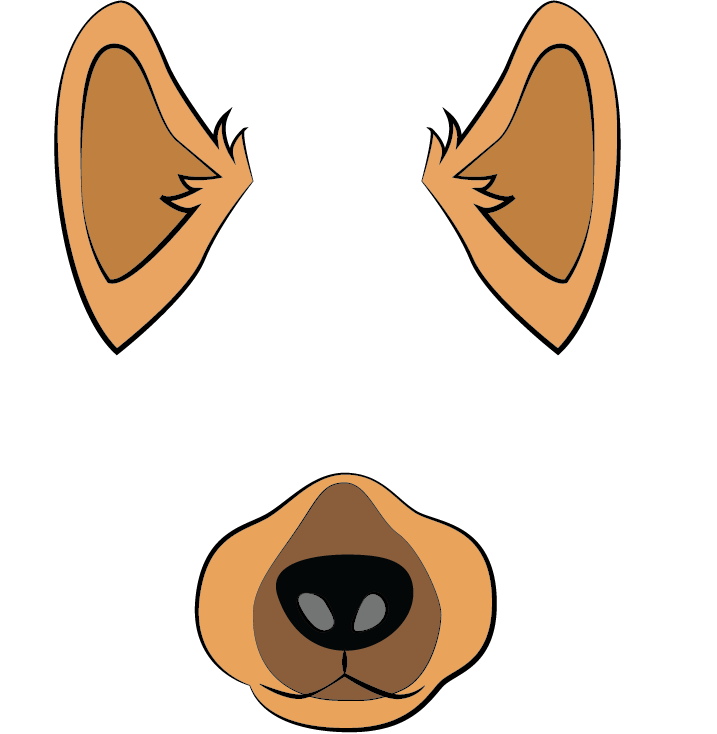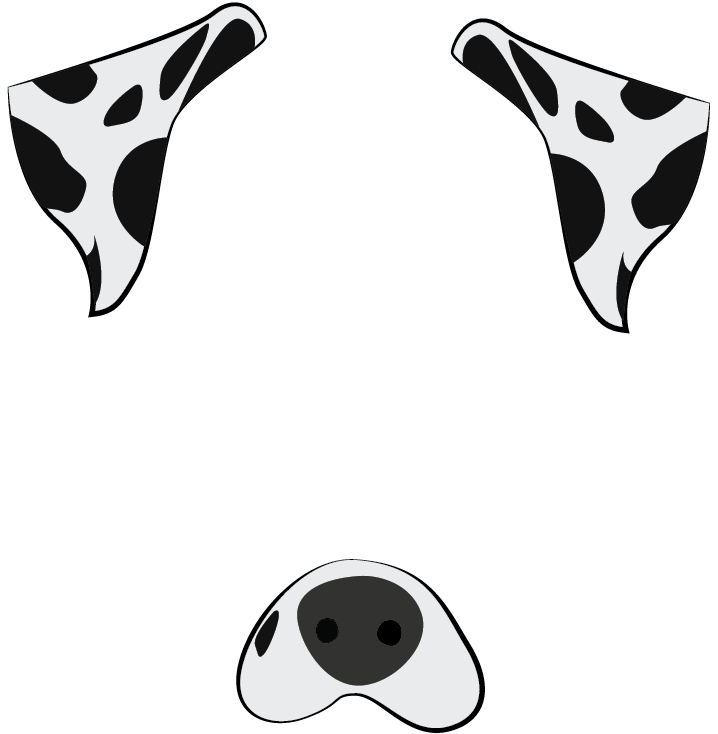Execute these commands to download both masks to your `assets` folder:

``wget -O assets/dalmation.png https://assets.digitalocean.com/articles/python3_dogfilter/E9ax7PI.png  # dalmation wget -O assets/sheepdog.png https://assets.digitalocean.com/articles/python3_dogfilter/HveFdkg.png  # sheepdog ``

Now let’s use the masks in our filter. Start by duplicating the `step_4_dog_mask.py` file:

``cp step_4_dog_mask.py step_8_dog_emotion_mask.py ``

Open the new Python script.

``nano step_8_dog_emotion_mask.py ``

Insert a new line at the top of the script to import the emotion predictor:

``from step_7_fer import get_image_to_emotion_predictor ... ``

Then, in the `main()` function, locate this line:

``    mask = cv2.imread('assets/dog.png') ``

Replace it with the following to load the new masks and aggregate all masks into a tuple:

``    mask0 = cv2.imread('assets/dog.png')     mask1 = cv2.imread('assets/dalmation.png')     mask2 = cv2.imread('assets/sheepdog.png')     masks = (mask0, mask1, mask2) ``

Add a line break, and then add this code to create the emotion predictor.

``     # get emotion predictor     predictor = get_image_to_emotion_predictor() ``

Your `main` function should now match the following:

``def main():     cap = cv2.VideoCapture(0)      # load mask     mask0 = cv2.imread('assets/dog.png')     mask1 = cv2.imread('assets/dalmation.png')     mask2 = cv2.imread('assets/sheepdog.png')     masks = (mask0, mask1, mask2)      # get emotion predictor     predictor = get_image_to_emotion_predictor()      # initialize front face classifier     ... ``

Next, locate these lines:

``             # apply mask             frame[y0: y1, x0: x1] = apply_mask(frame[y0: y1, x0: x1], mask) ``

Insert the following line below the `# apply mask` line to select the appropriate mask by using the predictor:

``            # apply mask             mask = masks[predictor(frame[y:y+h, x: x+w])]             frame[y0: y1, x0: x1] = apply_mask(frame[y0: y1, x0: x1], mask)  ``

The completed file should look like this:

``"""Test for face detection"""  from step_7_fer import get_image_to_emotion_predictor import numpy as np import cv2  def apply_mask(face: np.array, mask: np.array) -> np.array:     """Add the mask to the provided face, and return the face with mask."""     mask_h, mask_w, _ = mask.shape     face_h, face_w, _ = face.shape      # Resize the mask to fit on face     factor = min(face_h / mask_h, face_w / mask_w)     new_mask_w = int(factor * mask_w)     new_mask_h = int(factor * mask_h)     new_mask_shape = (new_mask_w, new_mask_h)     resized_mask = cv2.resize(mask, new_mask_shape)      # Add mask to face - ensure mask is centered     face_with_mask = face.copy()     non_white_pixels = (resized_mask < 250).all(axis=2)     off_h = int((face_h - new_mask_h) / 2)     off_w = int((face_w - new_mask_w) / 2)     face_with_mask[off_h: off_h+new_mask_h, off_w: off_w+new_mask_w][non_white_pixels] = \          resized_mask[non_white_pixels]      return face_with_mask  def main():      cap = cv2.VideoCapture(0)     # load mask     mask0 = cv2.imread('assets/dog.png')     mask1 = cv2.imread('assets/dalmation.png')     mask2 = cv2.imread('assets/sheepdog.png')     masks = (mask0, mask1, mask2)      # get emotion predictor     predictor = get_image_to_emotion_predictor()      # initialize front face classifier     cascade = cv2.CascadeClassifier("assets/haarcascade_frontalface_default.xml")      while True:         # Capture frame-by-frame         ret, frame = cap.read()         frame_h, frame_w, _ = frame.shape          # Convert to black-and-white         gray = cv2.cvtColor(frame, cv2.COLOR_BGR2GRAY)         blackwhite = cv2.equalizeHist(gray)          rects = cascade.detectMultiScale(             blackwhite, scaleFactor=1.3, minNeighbors=4, minSize=(30, 30),             flags=cv2.CASCADE_SCALE_IMAGE)          for x, y, w, h in rects:             # crop a frame slightly larger than the face             y0, y1 = int(y - 0.25*h), int(y + 0.75*h)             x0, x1 = x, x + w             # give up if the cropped frame would be out-of-bounds             if x0 < 0 or y0 < 0 or x1 > frame_w or y1 > frame_h:                 continue             # apply mask             mask = masks[predictor(frame[y:y+h, x: x+w])]             frame[y0: y1, x0: x1] = apply_mask(frame[y0: y1, x0: x1], mask)          # Display the resulting frame         cv2.imshow('frame', frame)         if cv2.waitKey(1) & 0xFF == ord('q'):             break      cap.release()     cv2.destroyAllWindows()  if __name__ == '__main__':     main() ``

Save and exit your editor. Now launch the script:

``python step_8_dog_emotion_mask.py ``

Now try it out! Smiling will register as “happy” and show the original dog. A neutral face or a frown will register as “sad” and yield the dalmation. A face of “surprise,” with a nice big jaw drop, will yield the sheepdog.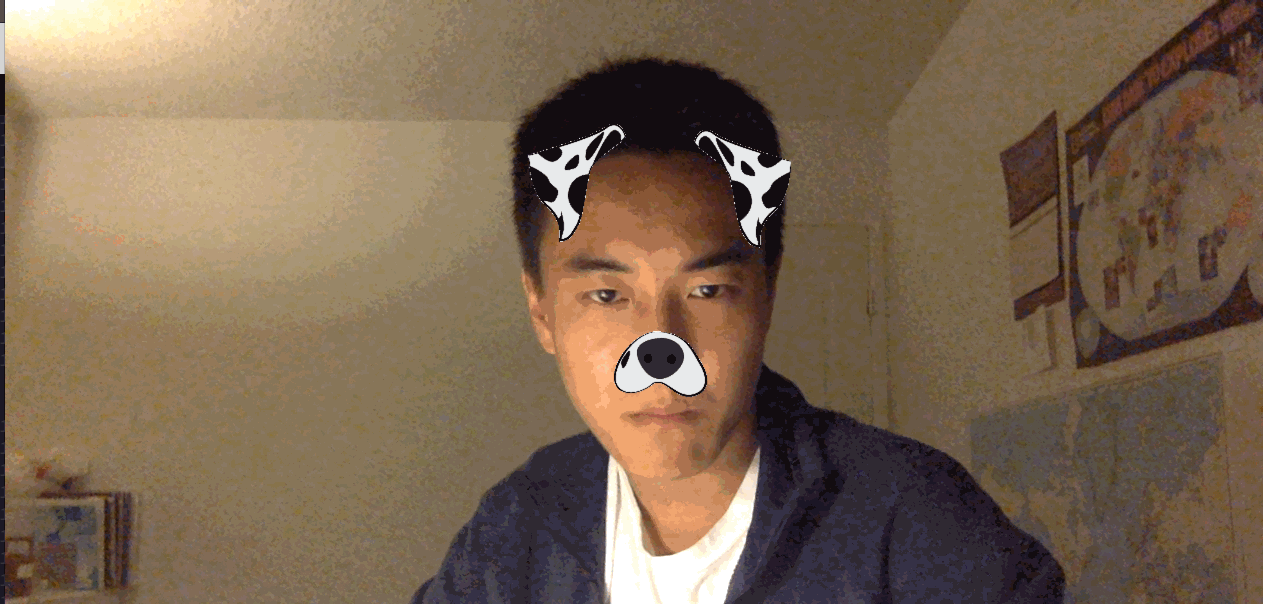This concludes our emotion-based dog filter and foray into computer vision.

## Conclusion

In this tutorial, you built a face detector and dog filter using computer vision and employed machine learning models to apply masks based on detected emotions.

Machine learning is widely applicable. However, it’s up to the practitioner to consider the ethical implications of each application when applying machine learning. The application you built in this tutorial was a fun exercise, but remember that you relied on OpenCV and an existing dataset to identify faces, rather than supplying your own data to train the models. The data and models used have significant impacts on how a program works.

For example, imagine a job search engine where the models were trained with data about candidates. such as race, gender, age, culture, first language, or other factors. And perhaps the developers trained a model that enforces sparsity, which ends up reducing the feature space to a subspace where gender explains most of the variance. As a result, the model influences candidate job searches and even company selection processes based primarily on gender. Now consider more complex situations where the model is less interpretable and you don’t know what a particular feature corresponds to. You can learn more about this in Equality of Opportunity in Machine Learning by Professor Moritz Hardt at UC Berkeley.

There can be an overwhelming magnitude of uncertainty in machine learning. To understand this randomness and complexity, you’ll have to develop both mathematical intuitions and probabilistic thinking skills. As a practitioner, it is up to you to dig into the theoretical underpinnings of machine learning.

DigitalOcean Community Tutorials

### Stack Abuse: Python for NLP: Sentiment Analysis with Scikit-Learn

This is the fifth article in the series of articles on NLP for Python. In my previous article, I explained how Python’s spaCy library can be used to perform parts of speech tagging and named entity recognition. In this article, I will demonstrate how to do sentiment analysis using Twitter data using the Scikit-Learn library.

Sentiment analysis refers to analyzing an opinion or feelings about something using data like text or images, regarding almost anything. Sentiment analysis helps companies in their decision-making process. For instance, if public sentiment towards a product is not so good, a company may try to modify the product or stop the production altogether in order to avoid any losses.

There are many sources of public sentiment e.g. public interviews, opinion polls, surveys, etc. However, with more and more people joining social media platforms, websites like Facebook and Twitter can be parsed for public sentiment.

In this article, we will see how we can perform sentiment analysis of text data.

### Problem Definition

Given tweets about six US airlines, the task is to predict whether a tweet contains positive, negative, or neutral sentiment about the airline. This is a typical supervised learning task where given a text string, we have to categorize the text string into predefined categories.

### Solution

To solve this problem, we will follow the typical machine learning pipeline. We will first import the required libraries and the dataset. We will then do exploratory data analysis to see if we can find any trends in the dataset. Next, we will perform text preprocessing to convert textual data to numeric data that can be used by a machine learning algorithm. Finally, we will use machine learning algorithms to train and test our sentiment analysis models.

#### Importing the Required Libraries

The first step as always is to import the required libraries:

``import numpy as np   import pandas as pd   import re   import nltk   import matplotlib.pyplot as plt   %matplotlib inline ``

Note: All the scripts in the article have been run using the Jupyter Notebook.

#### Importing the Dataset

To import the dataset, we will use the Pandas `read_csv` function, as shown below:

``data_source_url = "https://raw.githubusercontent.com/kolaveridi/kaggle-Twitter-US-Airline-Sentiment-/master/Tweets.csv"   airline_tweets = pd.read_csv(data_source_url)   ``

Let’s first see how the dataset looks like using the `head()` method:

``airline_tweets.head()   ``

The output looks like this:#### Data Analysis

Let’s explore the dataset a bit to see if we can find any trends. But before that, we will change the default plot size to have a better view of the plots. Execute the following script:

``plot_size = plt.rcParams["figure.figsize"]   print(plot_size)   print(plot_size)  plot_size = 8   plot_size = 6   plt.rcParams["figure.figsize"] = plot_size   ``

Let’s first see the number of tweets for each airline. We will plot a pie chart for that:

``airline_tweets.airline.value_counts().plot(kind='pie', autopct='%1.0f%%')   ``

In the output, you can see the percentage of public tweets for each airline. United Airline has the highest number of tweets i.e. 26%, followed by US Airways (20%).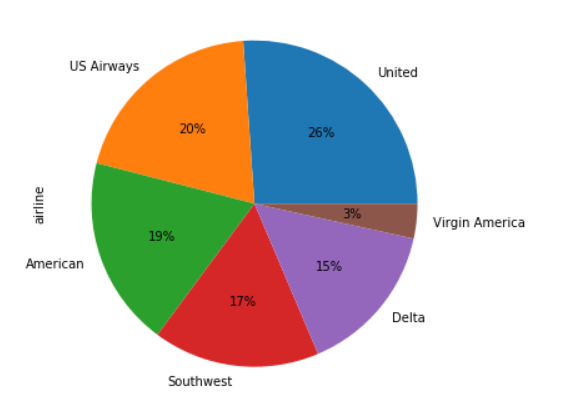Let’s now see the distribution of sentiments across all the tweets. Execute the following script:

``airline_tweets.airline_sentiment.value_counts().plot(kind='pie', autopct='%1.0f%%', colors=["red", "yellow", "green"])   ``

The output of the script above look likes this: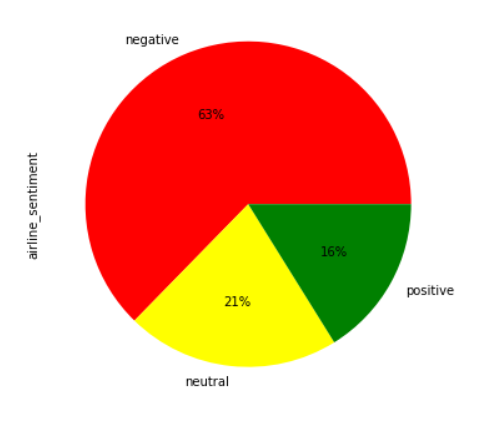From the output, you can see that the majority of the tweets are negative (63%), followed by neutral tweets (21%), and then the positive tweets (16%).

Next, let’s see the distribution of sentiment for each individual airline,

``airline_sentiment = airline_tweets.groupby(['airline', 'airline_sentiment']).airline_sentiment.count().unstack()   airline_sentiment.plot(kind='bar')   ``

The output looks like this:It is evident from the output that for almost all the airlines, the majority of the tweets are negative, followed by neutral and positive tweets. Virgin America is probably the only airline where the ratio of the three sentiments is somewhat similar.

Finally, let’s use the Seaborn library to view the average confidence level for the tweets belonging to three sentiment categories. Execute the following script:

``import seaborn as sns  sns.barplot(x='airline_sentiment', y='airline_sentiment_confidence' , data=airline_tweets)   ``

The output of the script above looks like this:From the output, you can see that the confidence level for negative tweets is higher compared to positive and neutral tweets.

Enough of the exploratory data analysis, our next step is to perform some preprocessing on the data and then convert the numeric data into text data as shown below.

#### Data Cleaning

Tweets contain many slang words and punctuation marks. We need to clean our tweets before they can be used for training the machine learning model. However, before cleaning the tweets, let’s divide our dataset into feature and label sets.

Our feature set will consist of tweets only. If we look at our dataset, the 11th column contains the tweet text. Note that the index of the column will be 10 since pandas columns follow zero-based indexing scheme where the first column is called 0th column. Our label set will consist of the sentiment of the tweet that we have to predict. The sentiment of the tweet is in the second column (index 1). To create a feature and a label set, we can use the `iloc` method off the pandas data frame.

Execute the following script:

``features = airline_tweets.iloc[:, 10].values   labels = airline_tweets.iloc[:, 1].values   ``

Once we divide the data into features and training set, we can preprocess data in order to clean it. To do so, we will use regular expressions. To study more about regular expressions, please take a look at this article on regular expressions.

``processed_features = []  for sentence in range(0, len(features)):       # Remove all the special characters     processed_feature = re.sub(r'\W', ' ', str(features[sentence]))      # remove all single characters     processed_feature= re.sub(r'\s+[a-zA-Z]\s+', ' ', processed_feature)      # Remove single characters from the start     processed_feature = re.sub(r'\^[a-zA-Z]\s+', ' ', processed_feature)       # Substituting multiple spaces with single space     processed_feature = re.sub(r'\s+', ' ', processed_feature, flags=re.I)      # Removing prefixed 'b'     processed_feature = re.sub(r'^b\s+', '', processed_feature)      # Converting to Lowercase     processed_feature = processed_feature.lower()      processed_features.append(processed_feature) ``

In the script above, we start by removing all the special characters from the tweets. The regular expression `re.sub(r'\W', ' ', str(features[sentence]))` does that.

Next, we remove all the single characters left as a result of removing the special character using the `re.sub(r'\s+[a-zA-Z]\s+', ' ', processed_feature)` regular expression. For instance, if we remove special character `'` from `Jack's` and replace it with space, we are left with `Jack s`. Here `s` has no meaning, so we remove it by replacing all single characters with a space.

However, if we replace all single characters with space, multiple spaces are created. Therefore, we replace all the multiple spaces with single spaces using `re.sub(r'\s+', ' ', processed_feature, flags=re.I)` regex. Furthermore, if your text string is in bytes format a character `b` is appended with the string. The above script removes that using the regex `re.sub(r'^b\s+', '', processed_feature)`.

Finally, the text is converted into lowercase using the `lower()` function.

#### Representing Text in Numeric Form

Statistical algorithms use mathematics to train machine learning models. However, mathematics only work with numbers. To make statistical algorithms work with text, we first have to convert text to numbers. To do so, three main approaches exist i.e. Bag of Words, TF-IDF and Word2Vec. In this section, we will discuss the bag of words and TF-IDF scheme.

##### Bag of Words

Bag of words scheme is the simplest way of converting text to numbers.

For instance, you have three documents:

• Doc1 = “I like to play football”
• Doc2 = “It is a good game”
• Doc3 = “I prefer football over rugby”

In the bag of words approach the first step is to create a vocabulary of all the unique words. For the above three documents, our vocabulary will be:

``Vocab = [I, like, to, play, football, it, is, a, good, game, prefer, over, rugby]   ``

The next step is to convert each document into a feature vector using the vocabulary. The length of each feature vector is equal to the length of the vocabulary. The frequency of the word in the document will replace the actual word in the vocabulary. If a word in the vocabulary is not found in the corresponding document, the document feature vector will have zero in that place. For instance, for Doc1, the feature vector will look like this:

``[1, 1, 1, 1, 1, 0, 0, 0, 0, 0, 0, 0, 0] ``
##### TF-IDF

In the bag of words approach, each word has the same weight. The idea behind the TF-IDF approach is that the words that occur less in all the documents and more in individual document contribute more towards classification.

TF-IDF is a combination of two terms. Term frequency and Inverse Document frequency. They can be calculated as:

``TF  = (Frequency of a word in the document)/(Total words in the document)  IDF = Log((Total number of docs)/(Number of docs containing the word))   ``
##### TF-IDF using the Scikit-Learn Library

Luckily for us, Python’s Scikit-Learn library contains the `TfidfVectorizer` class that can be used to convert text features into TF-IDF feature vectors. The following script performs this:

``from nltk.corpus import stopwords   from sklearn.feature_extraction.text import CountVectorizer  vectorizer = CountVectorizer(max_features=2500, min_df=7, max_df=0.8, stop_words=stopwords.words('english'))   X = vectorizer.fit_transform(processed_features).toarray()   ``

In the code above, we define that the `max_features` should be 2500, which means that it only uses the 2500 most frequently occurring words to create a bag of words feature vector. Words that occur less frequently are not very useful for classification.

Similarly, `max_df` specifies that only use those words that occur in a maximum of 80% of the documents. Words that occur in all documents are too common and are not very useful for classification. Similarly, `min-df` is set to 7 which shows that include words that occur in at least 7 documents.

#### Dividing Data into Training and Test Sets

In the previous section, we converted the data into the numeric form. As the last step before we train our algorithms, we need to divide our data into training and testing sets. The training set will be used to train the algorithm while the test set will be used to evaluate the performance of the machine learning model.

Execute the following code:

``from sklearn.model_selection import train_test_split  X_train, X_test, y_train, y_test = train_test_split(processed_features, labels, test_size=0.2, random_state=0)   ``

In the code above we use the `train_test_split` class from the `sklearn.model_selection` module to divide our data into training and testing set. The method takes the feature set as the first parameter, the label set as the second parameter, and a value for the `test_size` parameter. We specified a value of 0.2 for `test_size` which means that our data set will be split into two sets of 80% and 20% data. We will use the 80% dataset for training and 20% dataset for testing.

#### Training the Model

Once data is split into training and test set, machine learning algorithms can be used to learn from the training data. You can use any machine learning algorithm. However, we will use the Random Forest algorithm, owing to its ability to act upon non-normalized data.

The `sklearn.ensemble` module contains the `RandomForestClassifier` class that can be used to train the machine learning model using the random forest algorithm. To do so, we need to call the `fit` method on the `RandomForestClassifier` class and pass it our training features and labels, as parameters. Look at the following script:

``from sklearn.ensemble import RandomForestClassifier  text_classifier = RandomForestClassifier(n_estimators=200, random_state=0)   text_classifier.fit(X_train, y_train)   ``

#### Making Predictions and Evaluating the Model

Once the model has been trained, the last step is to make predictions on the model. To do so, we need to call the `predict` method on the object of the `RandomForestClassifier` class that we used for training. Look at the following script:

``predictions = text_classifier.predict(X_test)   ``

Finally, to evaluate the performance of the machine learning models, we can use classification metrics such as a confusion metrix, F1 measure, accuracy, etc.

To find the values for these metrics, we can use `classification_report`, `confusion_matrix`, and `accuracy_score` utilities from the `sklearn.metrics` library. Look a the following script:

``from sklearn.metrics import classification_report, confusion_matrix, accuracy_score  print(confusion_matrix(y_test,predictions))   print(classification_report(y_test,predictions))   print(accuracy_score(y_test, predictions))   ``

The output of the script above looks like this:

``[[1724  101   45]  [ 329  237   48]  [ 142   58  244]]               precision    recall  f1-score   support      negative       0.79      0.92      0.85      1870      neutral       0.60      0.39      0.47       614     positive       0.72      0.55      0.62       444     micro avg       0.75      0.75      0.75      2928    macro avg       0.70      0.62      0.65      2928 weighted avg       0.74      0.75      0.73      2928  0.7530737704918032   ``

From the output, you can see that our algorithm achieved an accuracy of 75.30.

### Conclusion

The sentiment analysis is one of the most commonly performed NLP tasks as it helps determine overall public opinion about a certain topic.

In this article, we saw how different Python libraries contribute to performing sentiment analysis. We performed an analysis of public tweets regarding six US airlines and achieved an accuracy of around 75%. I would recommend you to try and use some other machine learning algorithm such as logistic regression, SVM, or KNN and see if you can get better results.

Planet Python

### Introduction

When building modern, stateless applications, containerizing your application’s components is the first step in deploying and scaling on distributed platforms. If you have used Docker Compose in development, you will have modernized and containerized your application by:

• Extracting necessary configuration information from your code.
• Packaging your application for repeated use.

You will also have written service definitions that specify how your container images should run.

To run your services on a distributed platform like Kubernetes, you will need to translate your Compose service definitions to Kubernetes objects. This will allow you to scale your application with resiliency. One tool that can speed up the translation process to Kubernetes is kompose, a conversion tool that helps developers move Compose workflows to container orchestrators like Kubernetes or OpenShift.

In this tutorial, you will translate Compose services to Kubernetes objects using kompose. You will use the object definitions that kompose provides as a starting point and make adjustments to ensure that your setup will use Secrets, Services, and PersistentVolumeClaims in the way that Kubernetes expects. By the end of the tutorial, you will have a single-instance Node.js application with a MongoDB database running on a Kubernetes cluster. This setup will mirror the functionality of the code described in Containerizing a Node.js Application with Docker Compose and will be a good starting point to build out a production-ready solution that will scale with your needs.

## Step 1 — Installing kompose

To begin using kompose, navigate to the project’s GitHub Releases page, and copy the link to the current release (version 1.18.0 as of this writing). Paste this link into the following `curl` command to download the latest version of kompose:

``curl -L https://github.com/kubernetes/kompose/releases/download/v1.18.0/kompose-linux-amd64 -o kompose ``

For details about installing on non-Linux systems, please refer to the installation instructions.

Make the binary executable:

``chmod +x kompose ``

Move it to your `PATH`:

``sudo mv ./kompose /usr/local/bin/kompose ``

To verify that it has been installed properly, you can do a version check:

``kompose version ``

If the installation was successful, you will see output like the following:

``Output1.18.0 (06a2e56) ``

With `kompose` installed and ready to use, you can now clone the Node.js project code that you will be translating to Kubernetes.

## Step 2 — Cloning and Packaging the Application

To use our application with Kubernetes, we will need to clone the project code and package the application so that the `kubelet` service can pull the image.

Our first step will be to clone the node-mongo-docker-dev repository from the DigitalOcean Community GitHub account. This repository includes the code from the setup described in Containerizing a Node.js Application for Development With Docker Compose, which uses a demo Node.js application to demonstrate how to set up a development environment using Docker Compose. You can find more information about the application itself in the series From Containers to Kubernetes with Node.js.

Clone the repository into a directory called `node_project`:

``git clone https://github.com/do-community/node-mongo-docker-dev.git node_project ``

Navigate to the `node_project` directory:

``cd node_project ``

The `node_project` directory contains files and directories for a shark information application that works with user input. It has been modernized to work with containers: sensitive and specific configuration information has been removed from the application code and refactored to be injected at runtime, and the application’s state has been offloaded to a MongoDB database.

The project directory includes a `Dockerfile` with instructions for building the application image. Let’s build the image now so that you can push it to your Docker Hub account and use it in your Kubernetes setup.

Using the `docker build` command, build the image with the `-t` flag, which allows you to tag it with a memorable name. In this case, tag the image with your Docker Hub username and name it `node-kubernetes` or a name of your own choosing:

``docker build -t your_dockerhub_username/node-kubernetes . ``

The `.` in the command specifies that the build context is the current directory.

It will take a minute or two to build the image. Once it is complete, check your images:

``docker images ``

You will see the following output:

``OutputREPOSITORY                                TAG                 IMAGE ID            CREATED             SIZE your_dockerhub_username/node-kubernetes   latest              9c6f897e1fbc        3 seconds ago       90MB node                                      10-alpine           94f3c8956482        12 days ago         71MB ``

Next, log in to the Docker Hub account you created in the prerequisites:

``docker login -u your_dockerhub_username  ``

When prompted, enter your Docker Hub account password. Logging in this way will create a `~/.docker/config.json` file in your user’s home directory with your Docker Hub credentials.

Push the application image to Docker Hub with the `docker push` command. Remember to replace `your_dockerhub_username` with your own Docker Hub username:

``docker push your_dockerhub_username/node-kubernetes ``

You now have an application image that you can pull to run your application with Kubernetes. The next step will be to translate your application service definitions to Kubernetes objects.

## Step 3 — Translating Compose Services to Kubernetes Objects with kompose

Our Docker Compose file, here called `docker-compose.yaml`, lays out the definitions that will run our services with Compose. A service in Compose is a running container, and service definitions contain information about how each container image will run. In this step, we will translate these definitions to Kubernetes objects by using kompose to create `yaml` files. These files will contain specs for the Kubernetes objects that describe their desired state.

We will use these files to create different types of objects: Services, which will ensure that the Pods running our containers remain accessible; Deployments, which will contain information about the desired state of our Pods; a PersistentVolumeClaim to provision storage for our database data; a ConfigMap for environment variables injected at runtime; and a Secret for our application’s database user and password. Some of these definitions will be in the files kompose will create for us, and others we will need to create ourselves.

First, we will need to modify some of the definitions in our `docker-compose.yaml` file to work with Kubernetes. We will include a reference to our newly-built application image in our `nodejs` service definition and remove the bind mounts, volumes, and additional commands that we used to run the application container in development with Compose. Additionally, we’ll redefine both containers’ restart policies to be in line with the behavior Kubernetes expects.

Open the file with `nano` or your favorite editor:

``nano docker-compose.yaml ``

The current definition for the `nodejs` application service looks like this:

~/node_project/docker-compose.yaml
``... services:   nodejs:     build:       context: .       dockerfile: Dockerfile     image: nodejs     container_name: nodejs     restart: unless-stopped     env_file: .env     environment:       - MONGO_USERNAME=\$  MONGO_USERNAME       - MONGO_PASSWORD=\$  MONGO_PASSWORD       - MONGO_HOSTNAME=db       - MONGO_PORT=\$  MONGO_PORT       - MONGO_DB=\$  MONGO_DB      ports:       - "80:8080"     volumes:       - .:/home/node/app       - node_modules:/home/node/app/node_modules     networks:       - app-network     command: ./wait-for.sh db:27017 -- /home/node/app/node_modules/.bin/nodemon app.js ... ``

• Use your `node-kubernetes` image instead of the local `Dockerfile`.
• Change the container `restart` policy from `unless-stopped` to `always`.
• Remove the `volumes` list and the `command` instruction.

The finished service definition will now look like this:

~/node_project/docker-compose.yaml
``... services:   nodejs:     image: your_dockerhub_username/node-kubernetes     container_name: nodejs     restart: always     env_file: .env     environment:       - MONGO_USERNAME=\$  MONGO_USERNAME       - MONGO_PASSWORD=\$  MONGO_PASSWORD       - MONGO_HOSTNAME=db       - MONGO_PORT=\$  MONGO_PORT       - MONGO_DB=\$  MONGO_DB      ports:       - "80:8080"     networks:       - app-network ... ``

Next, scroll down to the `db` service definition. Here, change the `restart` policy for the service to `always` and remove the `.env` file. Instead of using values from the `.env` file, we will pass the values for our `MONGO_INITDB_ROOT_USERNAME` and `MONGO_INITDB_ROOT_PASSWORD` to the database container using the Secret we will create in Step 4.

The `db` service definition will now look like this:

~/node_project/docker-compose.yaml
``...   db:     image: mongo:4.1.8-xenial     container_name: db     restart: always     environment:       - MONGO_INITDB_ROOT_USERNAME=\$  MONGO_USERNAME       - MONGO_INITDB_ROOT_PASSWORD=\$  MONGO_PASSWORD     volumes:         - dbdata:/data/db        networks:       - app-network ...   ``

Finally, at the bottom of the file, remove the `node_modules` volumes from the top-level `volumes` key. The key will now look like this:

~/node_project/docker-compose.yaml
``... volumes:   dbdata: ``

Save and close the file when you are finished editing.

Before translating our service definitions, we will need to write the `.env` file that kompose will use to create the ConfigMap with our non-sensitive information. Please see Step 2 of Containerizing a Node.js Application for Development With Docker Compose for a longer explanation of this file.

In that tutorial, we added `.env` to our `.gitignore` file to ensure that it would not copy to version control. This means that it did not copy over when we cloned the node-mongo-docker-dev repository in Step 2 of this tutorial. We will therefore need to recreate it now.

Create the file:

``nano .env ``

kompose will use this file to create a ConfigMap for our application. However, instead of assigning all of the variables from the `nodejs` service definition in our Compose file, we will add only the `MONGO_DB` database name and the `MONGO_PORT`. We will assign the database username and password separately when we manually create a Secret object in Step 4.

Add the following port and database name information to the `.env` file. Feel free to rename your database if you would like:

~/node_project/.env
``MONGO_PORT=27017 MONGO_DB=sharkinfo ``

Save and close the file when you are finished editing.

You are now ready to create the files with your object specs. kompose offers multiple options for translating your resources. You can:

• Create `yaml` files based on the service definitions in your `docker-compose.yaml` file with `kompose convert`.
• Create Kubernetes objects directly with `kompose up`.
• Create a Helm chart with `kompose convert -c`.

For now, we will convert our service definitions to `yaml` files and then add to and revise the files kompose creates.

Convert your service definitions to `yaml` files with the following command:

``kompose convert ``

You can also name specific or multiple Compose files using the `-f` flag.

After you run this command, kompose will output information about the files it has created:

``OutputINFO Kubernetes file "nodejs-service.yaml" created  INFO Kubernetes file "db-deployment.yaml" created  INFO Kubernetes file "dbdata-persistentvolumeclaim.yaml" created  INFO Kubernetes file "nodejs-deployment.yaml" created  INFO Kubernetes file "nodejs-env-configmap.yaml" created  ``

These include `yaml` files with specs for the Node application Service, Deployment, and ConfigMap, as well as for the `dbdata` PersistentVolumeClaim and MongoDB database Deployment.

These files are a good starting point, but in order for our application’s functionality to match the setup described in Containerizing a Node.js Application for Development With Docker Compose we will need to make a few additions and changes to the files kompose has generated.

## Step 4 — Creating Kubernetes Secrets

In order for our application to function in the way we expect, we will need to make a few modifications to the files that kompose has created. The first of these changes will be generating a Secret for our database user and password and adding it to our application and database Deployments. Kubernetes offers two ways of working with environment variables: ConfigMaps and Secrets. kompose has already created a ConfigMap with the non-confidential information we included in our `.env` file, so we will now create a Secret with our confidential information: our database username and password.

The first step in manually creating a Secret will be to convert your username and password to base64, an encoding scheme that allows you to uniformly transmit data, including binary data.

``echo -n 'your_database_username' | base64 ``

Note down the value you see in the output.

``echo -n 'your_database_password' | base64 ``

Take note of the value in the output here as well.

Open a file for the Secret:

``nano secret.yaml ``

Note: Kubernetes objects are typically defined using YAML, which strictly forbids tabs and requires two spaces for indentation. If you would like to check the formatting of any of your `yaml` files, you can use a linter or test the validity of your syntax using `kubectl create` with the `--dry-run` and `--validate` flags:

``kubectl create -f your_yaml_file.yaml --dry-run --validate=true ``

In general, it is a good idea to validate your syntax before creating resources with `kubectl`.

Add the following code to the file to create a Secret that will define your `MONGO_USERNAME` and `MONGO_PASSWORD` using the encoded values you just created. Be sure to replace the dummy values here with your encoded username and password:

~/node_project/secret.yaml
``apiVersion: v1 kind: Secret metadata:   name: mongo-secret data:   MONGO_USERNAME: your_encoded_username   MONGO_PASSWORD: your_encoded_password ``

We have named the Secret object `mongo-secret`, but you are free to name it anything you would like.

Save and close this file when you are finished editing. As you did with your `.env` file, be sure to add `secret.yaml` to your `.gitignore` file to keep it out of version control.

With `secret.yaml` written, our next step will be to ensure that our application and database Pods both use the values we added to the file. Let’s start by adding references to the Secret to our application Deployment.

Open the file called `nodejs-deployment.yaml`:

``nano nodejs-deployment.yaml ``

The file’s container specifications include the following environment variables defined under the `env` key:

~/node_project/nodejs-deployment.yaml
``apiVersion: extensions/v1beta1 kind: Deployment ...     spec:       containers:       - env:         - name: MONGO_DB           valueFrom:             configMapKeyRef:               key: MONGO_DB               name: nodejs-env         - name: MONGO_HOSTNAME           value: db         - name: MONGO_PASSWORD         - name: MONGO_PORT           valueFrom:             configMapKeyRef:               key: MONGO_PORT               name: nodejs-env         - name: MONGO_USERNAME ``

We will need to add references to our Secret to the `MONGO_USERNAME` and `MONGO_PASSWORD` variables listed here, so that our application will have access to those values. Instead of including a `configMapKeyRef` key to point to our `nodejs-env` ConfigMap, as is the case with the values for `MONGO_DB` and `MONGO_PORT`, we’ll include a `secretKeyRef` key to point to the values in our `mongo-secret` secret.

Add the following Secret references to the `MONGO_USERNAME` and `MONGO_PASSWORD` variables:

~/node_project/nodejs-deployment.yaml
``apiVersion: extensions/v1beta1 kind: Deployment ...     spec:       containers:       - env:         - name: MONGO_DB           valueFrom:             configMapKeyRef:               key: MONGO_DB               name: nodejs-env         - name: MONGO_HOSTNAME           value: db         - name: MONGO_PASSWORD           valueFrom:             secretKeyRef:               name: mongo-secret               key: MONGO_PASSWORD         - name: MONGO_PORT           valueFrom:             configMapKeyRef:               key: MONGO_PORT               name: nodejs-env         - name: MONGO_USERNAME           valueFrom:             secretKeyRef:               name: mongo-secret               key: MONGO_USERNAME ``

Save and close the file when you are finished editing.

Next, we’ll add the same values to the `db-deployment.yaml` file.

Open the file for editing:

``nano db-deployment.yaml ``

In this file, we will add references to our Secret for following variable keys: `MONGO_INITDB_ROOT_USERNAME` and `MONGO_INITDB_ROOT_PASSWORD`. The `mongo` image makes these variables available so that you can modify the initialization of your database instance. `MONGO_INITDB_ROOT_USERNAME` and `MONGO_INITDB_ROOT_PASSWORD` together create a `root` user in the `admin` authentication database and ensure that authentication is enabled when the database container starts.

Using the values we set in our Secret ensures that we will have an application user with `root` privileges on the database instance, with access to all of the administrative and operational privileges of that role. When working in production, you will want to create a dedicated application user with appropriately scoped privileges.

Under the `MONGO_INITDB_ROOT_USERNAME` and `MONGO_INITDB_ROOT_PASSWORD` variables, add references to the Secret values:

~/node_project/db-deployment.yaml
``apiVersion: extensions/v1beta1 kind: Deployment ...     spec:       containers:       - env:         - name: MONGO_INITDB_ROOT_PASSWORD           valueFrom:             secretKeyRef:               name: mongo-secret               key: MONGO_PASSWORD                 - name: MONGO_INITDB_ROOT_USERNAME           valueFrom:             secretKeyRef:               name: mongo-secret               key: MONGO_USERNAME         image: mongo:4.1.8-xenial ... ``

Save and close the file when you are finished editing.

With your Secret in place, you can move on to creating your database Service and ensuring that your application container only attempts to connect to the database once it is fully set up and initialized.

## Step 5 — Creating the Database Service and an Application Init Container

Now that we have our Secret, we can move on to creating our database Service and an Init Container that will poll this Service to ensure that our application only attempts to connect to the database once the database startup tasks, including creating the `MONGO_INITDB` user and password, are complete.

For a discussion of how to implement this functionality in Compose, please see Step 4 of Containerizing a Node.js Application for Development with Docker Compose.

Open a file to define the specs for the database Service:

``nano db-service.yaml   ``

Add the following code to the file to define the Service:

~/node_project/db-service.yaml
``apiVersion: v1 kind: Service metadata:   annotations:      kompose.cmd: kompose convert     kompose.version: 1.18.0 (06a2e56)   creationTimestamp: null   labels:     io.kompose.service: db   name: db spec:   ports:   - port: 27017     targetPort: 27017   selector:     io.kompose.service: db status:   loadBalancer: {} ``

The `selector` that we have included here will match this Service object with our database Pods, which have been defined with the label `io.kompose.service: db` by kompose in the `db-deployment.yaml` file. We’ve also named this service `db`.

Save and close the file when you are finished editing.

Next, let’s add an Init Container field to the `containers` array in `nodejs-deployment.yaml`. This will create an Init Container that we can use to delay our application container from starting until the `db` Service has been created with a Pod that is reachable. This is one of the possible uses for Init Containers; to learn more about other use cases, please see the official documentation.

Open the `nodejs-deployment.yaml` file:

``nano nodejs-deployment.yaml ``

Within the Pod spec and alongside the `containers` array, we are going to add an `initContainers` field with a container that will poll the `db` Service.

Add the following code below the `ports` and `resources` fields and above the `restartPolicy` in the `nodejs` `containers` array:

~/node_project/nodejs-deployment.yaml
``apiVersion: extensions/v1beta1 kind: Deployment ...     spec:       containers:       ...         name: nodejs         ports:         - containerPort: 8080         resources: {}       initContainers:       - name: init-db         image: busybox         command: ['sh', '-c', 'until nc -z db:27017; do echo waiting for db; sleep 2; done;']       restartPolicy: Always ...                ``

This Init Container uses the BusyBox image, a lightweight image that includes many UNIX utilities. In this case, we’ll use the `netcat` utility to poll whether or not the Pod associated with the `db` Service is accepting TCP connections on port `27017`.

This container `command` replicates the functionality of the `wait-for` script that we removed from our `docker-compose.yaml` file in Step 3. For a longer discussion of how and why our application used the `wait-for` script when working with Compose, please see Step 4 of Containerizing a Node.js Application for Development with Docker Compose.

Init Containers run to completion; in our case, this means that our Node application container will not start until the database container is running and accepting connections on port `27017`. The `db` Service definition allows us to guarantee this functionality regardless of the exact location of the database container, which is mutable.

Save and close the file when you are finished editing.

With your database Service created and your Init Container in place to control the startup order of your containers, you can move on to checking the storage requirements in your PersistentVolumeClaim and exposing your application service using a LoadBalancer.

## Step 6 — Modifying the PersistentVolumeClaim and Exposing the Application Frontend

Before running our application, we will make two final changes to ensure that our database storage will be provisioned properly and that we can expose our application frontend using a LoadBalancer.

First, let’s modify the `storage` `resource` defined in the PersistentVolumeClaim that kompose created for us. This Claim allows us to dynamically provision storage to manage our application’s state.

To work with PersistentVolumeClaims, you must have a StorageClass created and configured to provision storage resources. In our case, because we are working with DigitalOcean Kubernetes, our default StorageClass `provisioner` is set to `dobs.csi.digitalocean.com`DigitalOcean Block Storage.

We can check this by typing:

``kubectl get storageclass ``

If you are working with a DigitalOcean cluster, you will see the following output:

``OutputNAME                         PROVISIONER                 AGE do-block-storage (default)   dobs.csi.digitalocean.com   76m ``

If you are not working with a DigitalOcean cluster, you will need to create a StorageClass and configure a `provisioner` of your choice. For details about how to do this, please see the official documentation.

When kompose created `dbdata-persistentvolumeclaim.yaml`, it set the `storage` `resource` to a size that does not meet the minimum size requirements of our `provisioner`. We will therefore need to modify our PersistentVolumeClaim to use the minimum viable DigitalOcean Block Storage unit: 1GB. Please feel free to modify this to meet your storage requirements.

Open `dbdata-persistentvolumeclaim.yaml`:

``nano dbdata-persistentvolumeclaim.yaml ``

Replace the `storage` value with `1Gi`:

~/node_project/dbdata-persistentvolumeclaim.yaml
``apiVersion: v1 kind: PersistentVolumeClaim metadata:   creationTimestamp: null   labels:     io.kompose.service: dbdata   name: dbdata spec:   accessModes:   - ReadWriteOnce   resources:     requests:       storage: 1Gi status: {} ``

Also note the `accessMode`: `ReadWriteOnce` means that the volume provisioned as a result of this Claim will be read-write only by a single node. Please see the documentation for more information about different access modes.

Save and close the file when you are finished.

Next, open `nodejs-service.yaml`:

``nano nodejs-service.yaml ``

We are going to expose this Service externally using a DigitalOcean Load Balancer. If you are not using a DigitalOcean cluster, please consult the relevant documentation from your cloud provider for information about their load balancers. Alternatively, you can follow the official Kubernetes documentation on setting up a highly available cluster with `kubeadm`, but in this case you will not be able to use PersistentVolumeClaims to provision storage.

Within the Service spec, specify `LoadBalancer` as the Service `type`:

~/node_project/nodejs-service.yaml
``apiVersion: v1 kind: Service ... spec:   type: LoadBalancer   ports: ... ``

When we create the `nodejs` Service, a load balancer will be automatically created, providing us with an external IP where we can access our application.

Save and close the file when you are finished editing.

With all of our files in place, we are ready to start and test the application.

## Step 7 — Starting and Accessing the Application

It’s time to create our Kubernetes objects and test that our application is working as expected.

To create the objects we’ve defined, we’ll use `kubectl create` with the `-f` flag, which will allow us to specify the files that kompose created for us, along with the files we wrote. Run the following command to create the Node application and MongoDB database Services and Deployments, along with your Secret, ConfigMap, and PersistentVolumeClaim:

``kubectl create -f nodejs-service.yaml,nodejs-deployment.yaml,nodejs-env-configmap.yaml,db-service.yaml,db-deployment.yaml,dbdata-persistentvolumeclaim.yaml,secret.yaml ``

You will see the following output indicating that the objects have been created:

``Outputservice/nodejs created deployment.extensions/nodejs created configmap/nodejs-env created service/db created deployment.extensions/db created persistentvolumeclaim/dbdata created secret/mongo-secret created ``

To check that your Pods are running, type:

``kubectl get pods ``

You don’t need to specify a Namespace here, since we have created our objects in the `default` Namespace. If you are working with multiple Namespaces, be sure to include the `-n` flag when running this command, along with the name of your Namespace.

You will see the following output while your `db` container is starting and your application Init Container is running:

``OutputNAME                      READY   STATUS              RESTARTS   AGE db-679d658576-kfpsl       0/1     ContainerCreating   0          10s nodejs-6b9585dc8b-pnsws   0/1     Init:0/1            0          10s ``

Once that container has run and your application and database containers have started, you will see this output:

``OutputNAME                      READY   STATUS    RESTARTS   AGE db-679d658576-kfpsl       1/1     Running   0          54s nodejs-6b9585dc8b-pnsws   1/1     Running   0          54s ``

The `Running` `STATUS` indicates that your Pods are bound to nodes and that the containers associated with those Pods are running. `READY` indicates how many containers in a Pod are running. For more information, please consult the documentation on Pod lifecycles.

Note:
If you see unexpected phases in the `STATUS` column, remember that you can troubleshoot your Pods with the following commands:

``kubectl describe pods your_pod kubectl logs your_pod ``

With your containers running, you can now access the application. To get the IP for the LoadBalancer, type:

``kubectl get svc ``

You will see the following output:

``OutputNAME         TYPE           CLUSTER-IP       EXTERNAL-IP      PORT(S)        AGE db           ClusterIP      10.245.189.250   <none>           27017/TCP      93s kubernetes   ClusterIP      10.245.0.1       <none>           443/TCP        25m12s nodejs       LoadBalancer   10.245.15.56     your_lb_ip       80:30729/TCP   93s ``

The `EXTERNAL_IP` associated with the `nodejs` service is the IP address where you can access the application. If you see a `<pending>` status in the `EXTERNAL_IP` column, this means that your load balancer is still being created.

Once you see an IP in that column, navigate to it in your browser: `http://your_lb_ip`.

You should see the following landing page: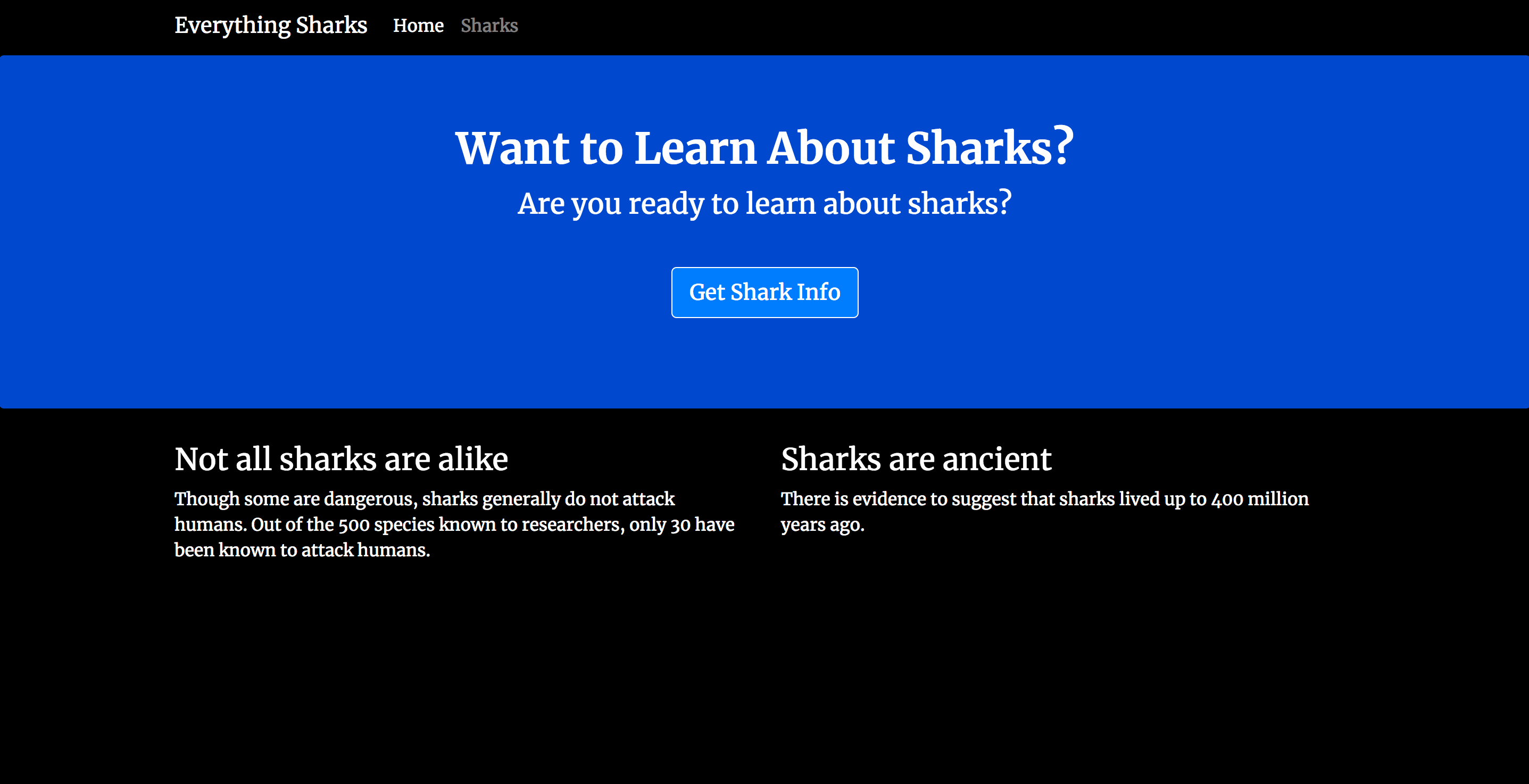Click on the Get Shark Info button. You will see a page with an entry form where you can enter a shark name and a description of that shark’s general character: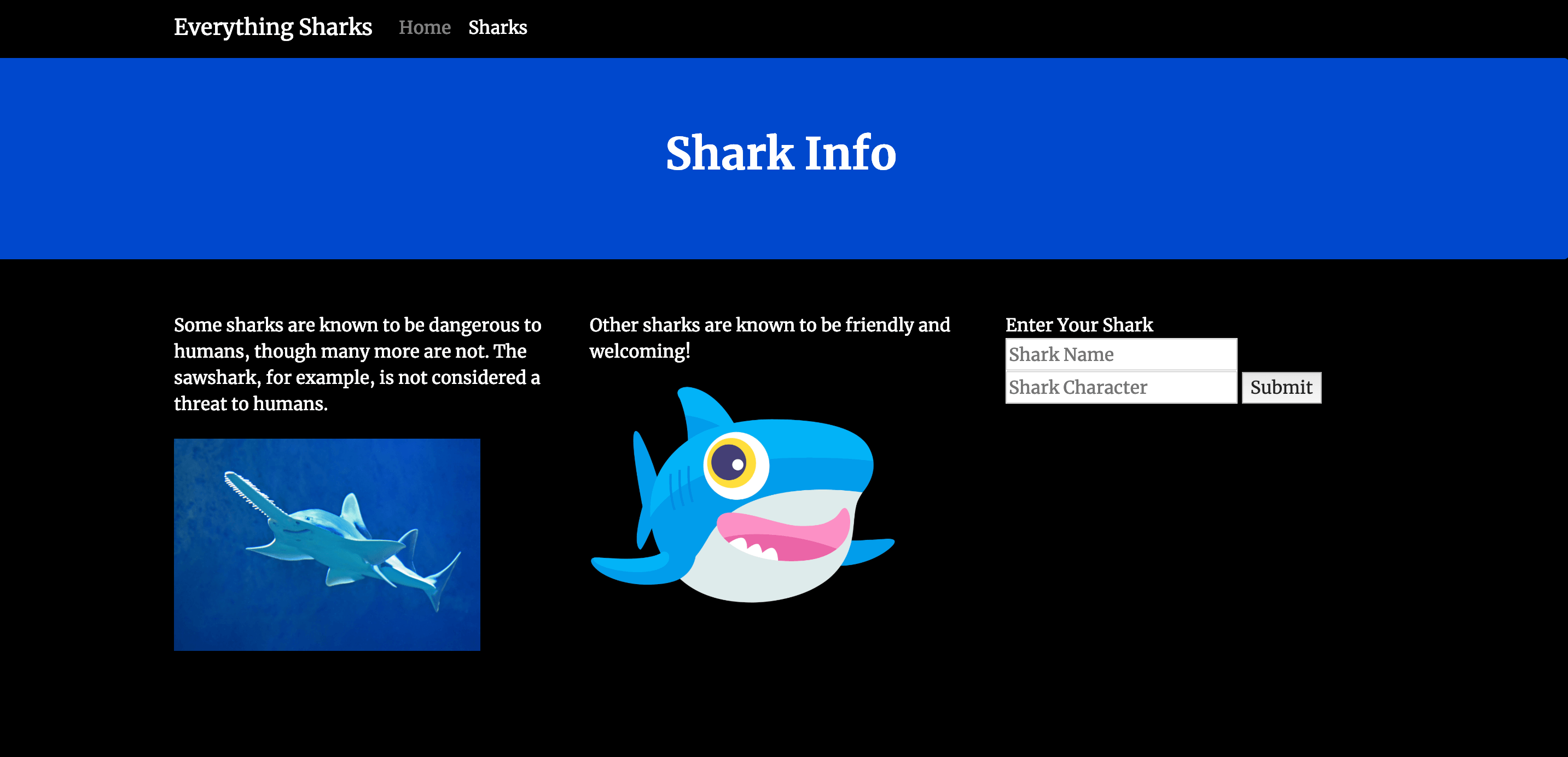In the form, add a shark of your choosing. To demonstrate, we will add `Megalodon Shark` to the Shark Name field, and `Ancient` to the Shark Character field: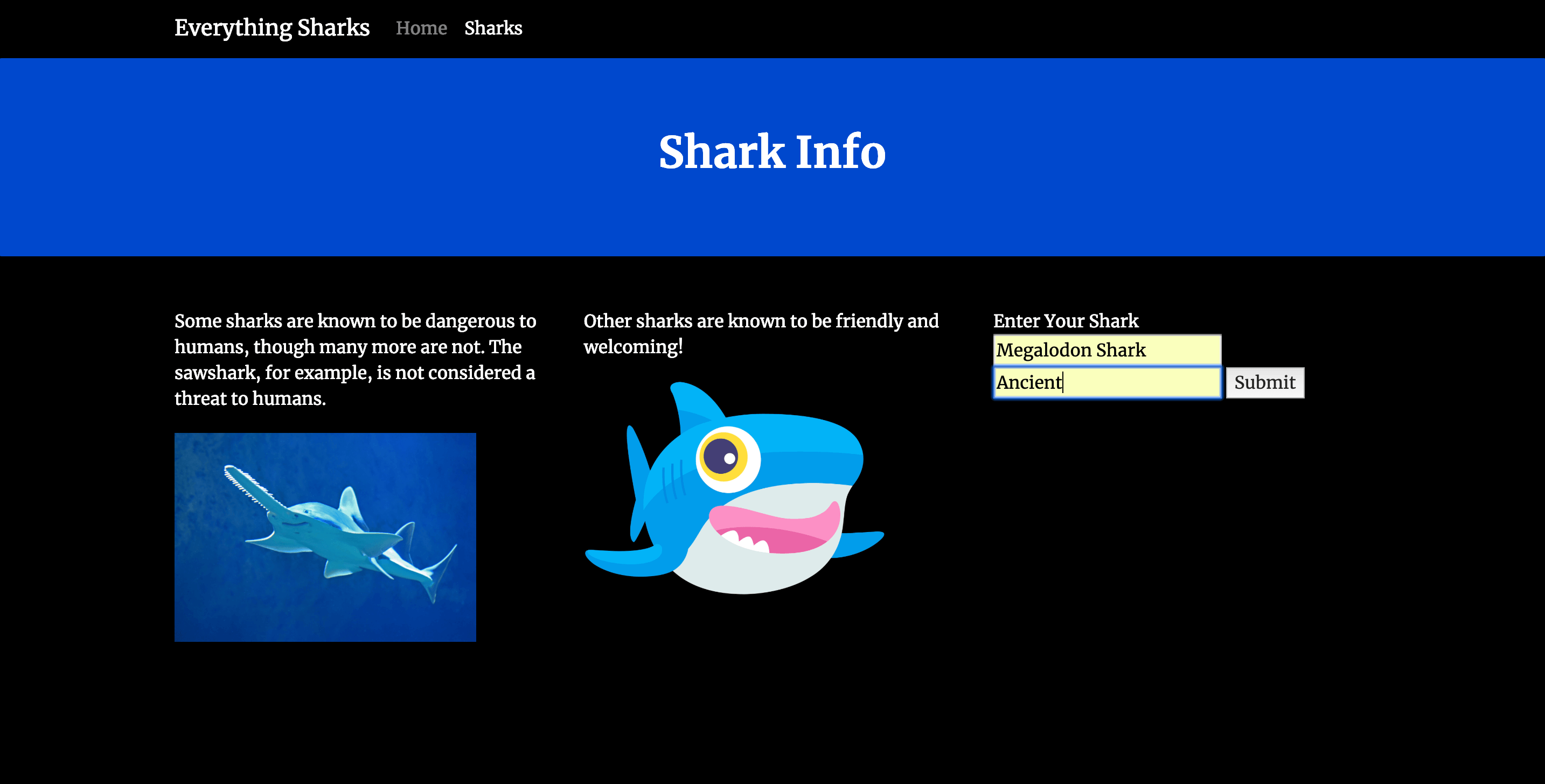Click on the Submit button. You will see a page with this shark information displayed back to you: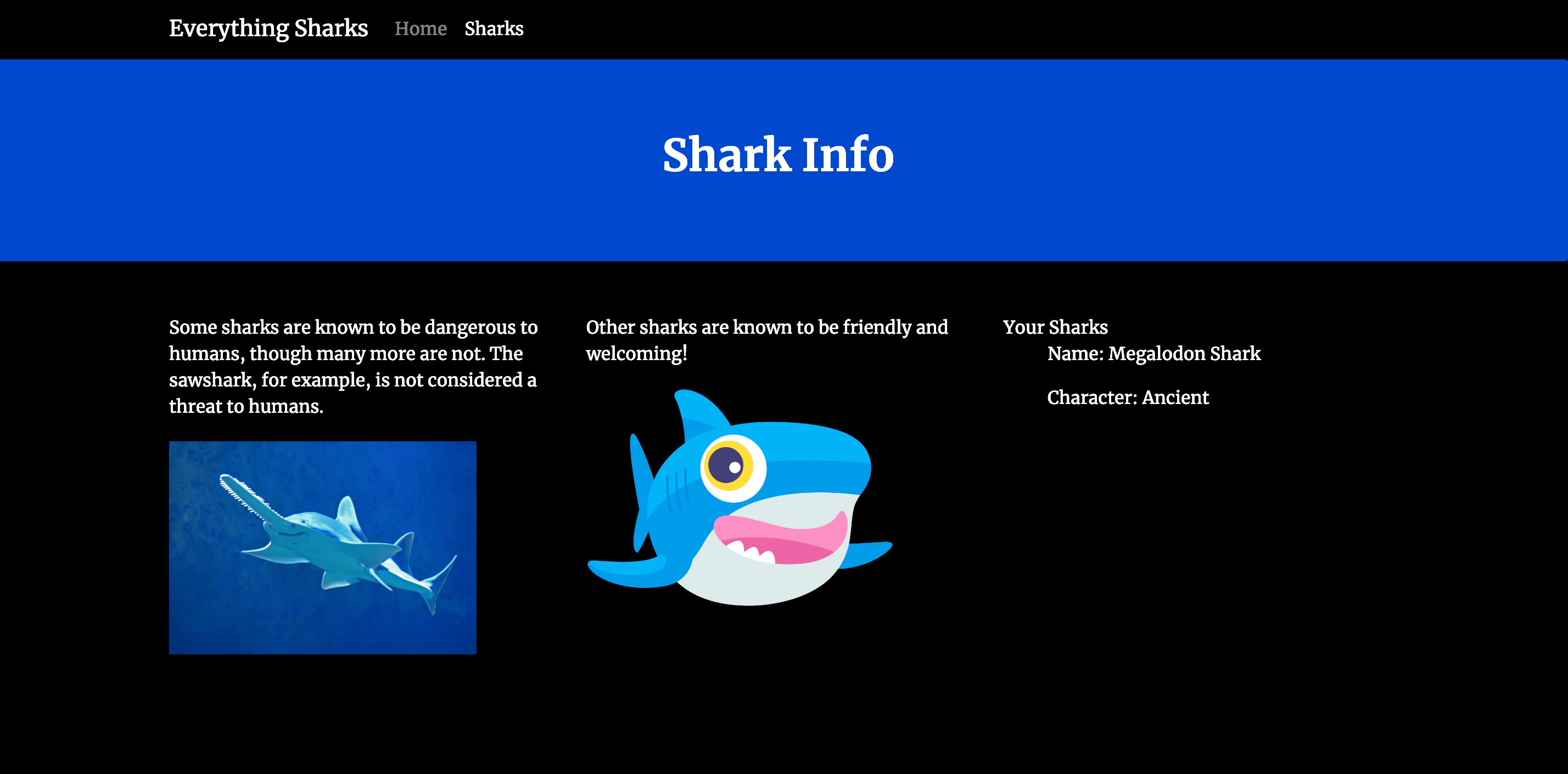You now have a single instance setup of a Node.js application with a MongoDB database running on a Kubernetes cluster.

## Conclusion

The files you have created in this tutorial are a good starting point to build from as you move toward production. As you develop your application, you can work on implementing the following:

DigitalOcean Community Tutorials

### Portals for Tableau 101: Analyzing Your Google Analytics or Matomo Traffic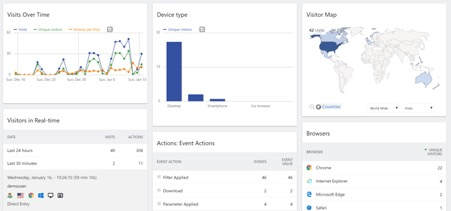If you have a portal for Tableau, then you’re certainly interested in analytics, but are you interested enough to get analytics on your analytics solution? I know I am! Fortunately, your portal makes it is easy to add in Google Analytics or Matomo to begin tracking your traffic. It’s worth noting that Google Analytics and Matomo will track internal and external-facing sites, so whether you have a client-facing portal or one just for internal use, this functionality will be useful. This post will review how to set up each and begin viewing all that beautiful data:Once you land on the account page for your portal, copy the Tracking ID: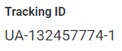Navigate to the backend of your portal, choose Settings from the top menu, then choose Portal Settings from the left menu:Enter the tracking ID in the box under Google Analytics Tracking ID:## Setting up Matomo (Formerly Piwik) – Cloud ServiceFill out the form with your portal URL and click the big green button:When you receive the confirmation email, log in with the credentials they give and note the URL. It’ll look something like https://yourPortal.matomo.cloud. This will be what you enter into Piwik/Matomo Server URL on the portal backend:If this is your initial use of Matomo, your site ID will automatically be 1. If you are tracking several sites already and the portal is an addition, go to your Matomo account site (https://yourPortal.matomo.cloud usually), and navigate to your settings (the typical settings gear logo on the top right of your screen). You can select Manage under Websites in the left menu, and it’ll display all your sites and all the site IDs. Find the ID for the portal and enter it into Piwik/Matomo Site ID:The Matomo cloud service unfortunately isn’t free forever, but it does allow you to skip configuring and managing the on-premise service on your server. Find the pricing for Matomo’s cloud service here.

## Matomo – On-Premise

If your business prefers to keep all analytics on a server on premises or self-managed in a cloud service like AWS, Matomo also offers their web analytics solution for free and downloadable from here:Here’s a thorough guide on how to install and configure Matomo on your server.

Once Matomo is installed, we follow the same process as the cloud service. Find your server URL (usually //matomo.site.com/) and specific site ID, and enter them into the backend of your portal under Settings > Portal Settings > Piwik/Matomo Server URL and Piwik/Matomo Site ID:The post Portals for Tableau 101: Analyzing Your Google Analytics or Matomo Traffic appeared first on InterWorks.

InterWorks